Updated date:

# How to Understand Electricity: Volts, Amps, Watts and Electrical Appliances

Eugene is a qualified control/instrumentation engineer Bsc (Eng) and has worked as a developer of electronics & software for SCADA systems.

## A Beginner's Guide to Electricity

This guide for consumers and students explains all about volts, amps and watts and how they apply to home appliances and circuits. The equations are really quite simple, and you'll find some examples on how to apply them to home appliances.

Please share a link to this guide on Pinterest, Facebook or other social media if you find it useful.

## Electricity 101: Understanding the Basics

In this tutorial, you'll learn about:

• volts, watts, amps
• power consumption of appliances and kilowatt hours (kWh).
• Ohm's law and resistance
• resistivity and how it affects the resistance of a material
• fuses and how they protect wiring and appliances
• how electricity is produced
• devices used to measure voltage, current and resistance
• the effects of electric and magnetic fields
• conductors, insulators and superconductors
• the basics of AC and DC
• arcs and sparks
• power supplies and voltage regulation
• tracking electricity usage in the home

## What is Electricity and an Electric Current?

An electric current is a flow of electrons in a conductor. All matter is made from basic building blocks called atoms. A simplistic model of an atom, known as the Rutherford–Bohr model or Bohr model or Bohr diagram has a central nucleus made up of particles called protons and neutrons. The nucleus is surrounded by orbitals containing electrons. In some materials such as metals, electrons are bound loosely to the nucleus so they can detach and move when a voltage is applied. These materials are known as conductors and can conduct electricity. The flow of electrons is called a current.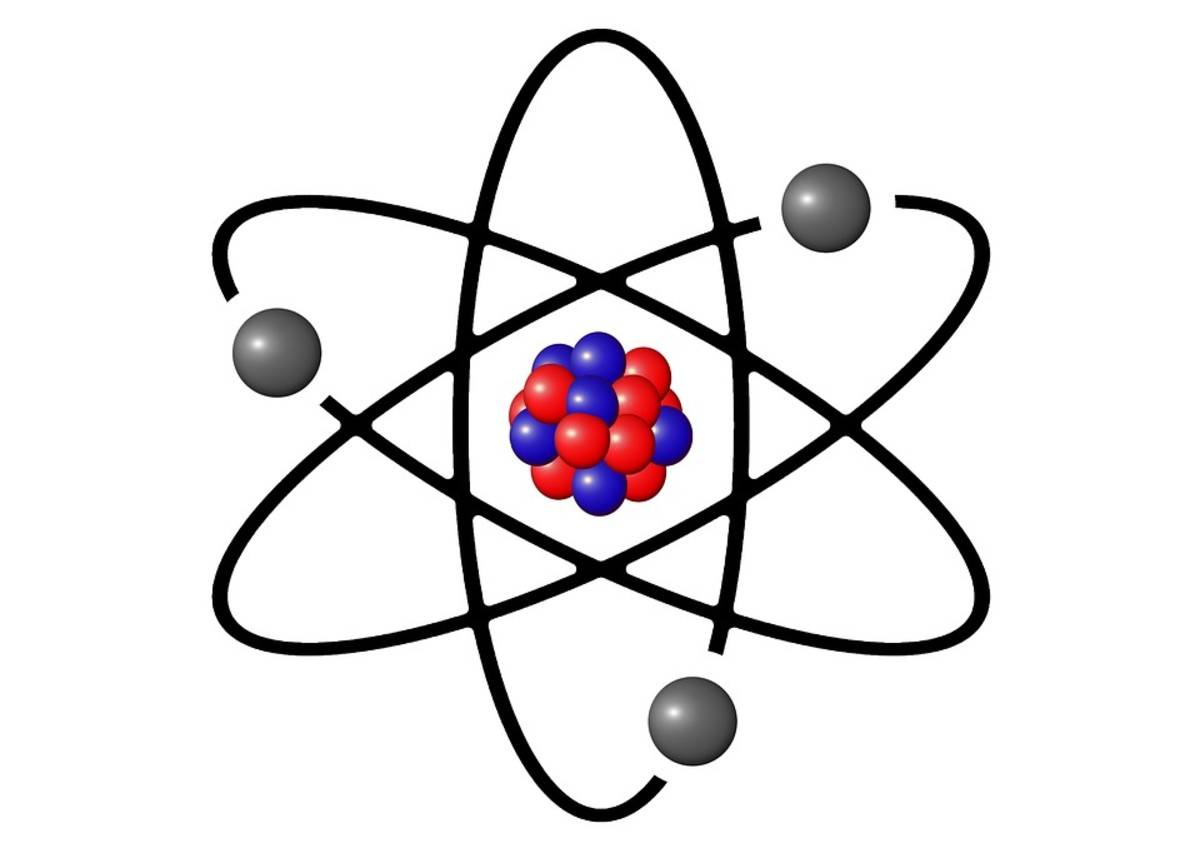Conceptual image of atom with protons and neutrons in the central nucleus and electrons in outer orbitalsGeralt, public domain image via Pixabay.com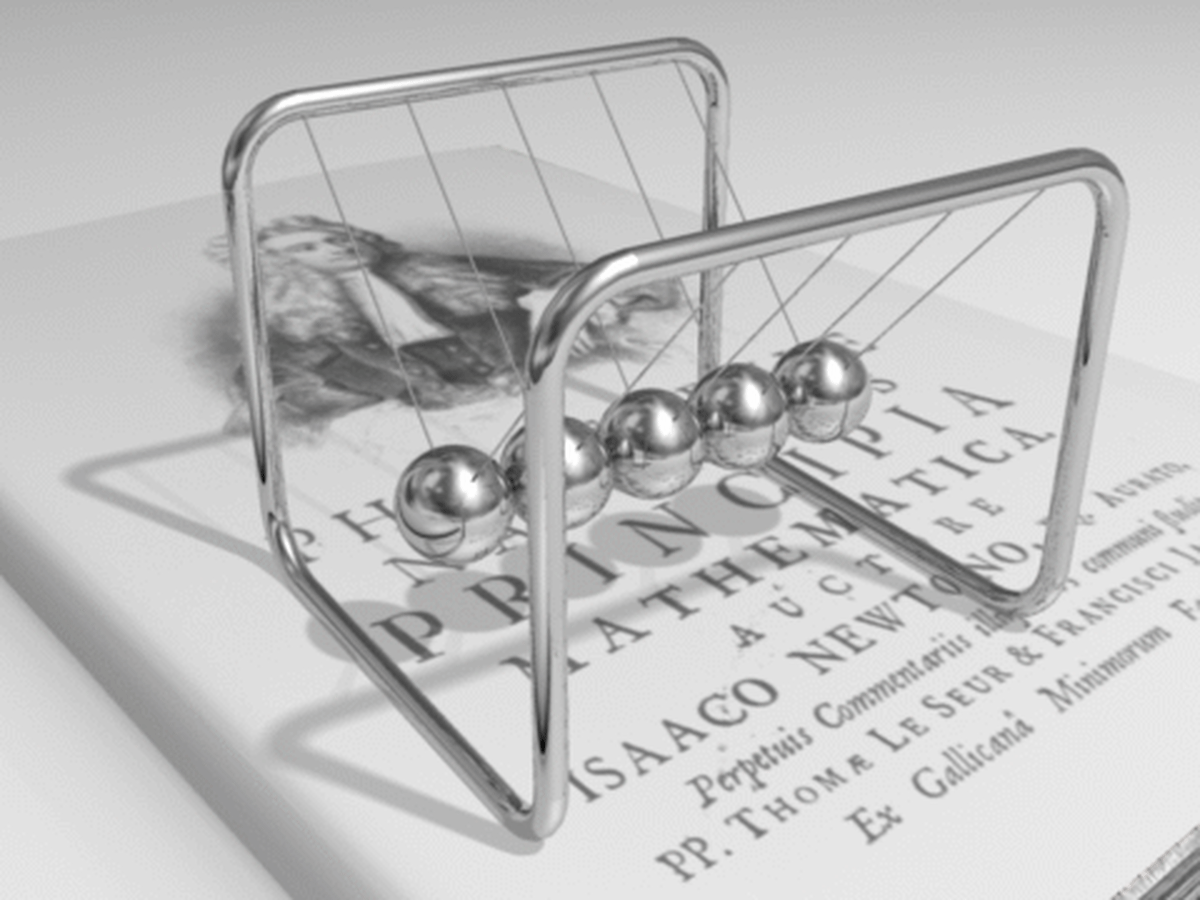In reality, electrons don't actually move from one end of a conductor to another. Instead they transfer energy between each other just like the steel balls in this Newton's cradle, while moving negligibly themselves.DemondeLuxe, Creative Commons Image 3.0 unported

## How Does Current Flow in a Circuit?

In the photo below, an AA cell powers a torch bulb. Current first flows out the top of the battery, through the wire and bulb and then returns via the bottom wire. So it always flows in a loop and two wires are needed to connect the voltage source to the load.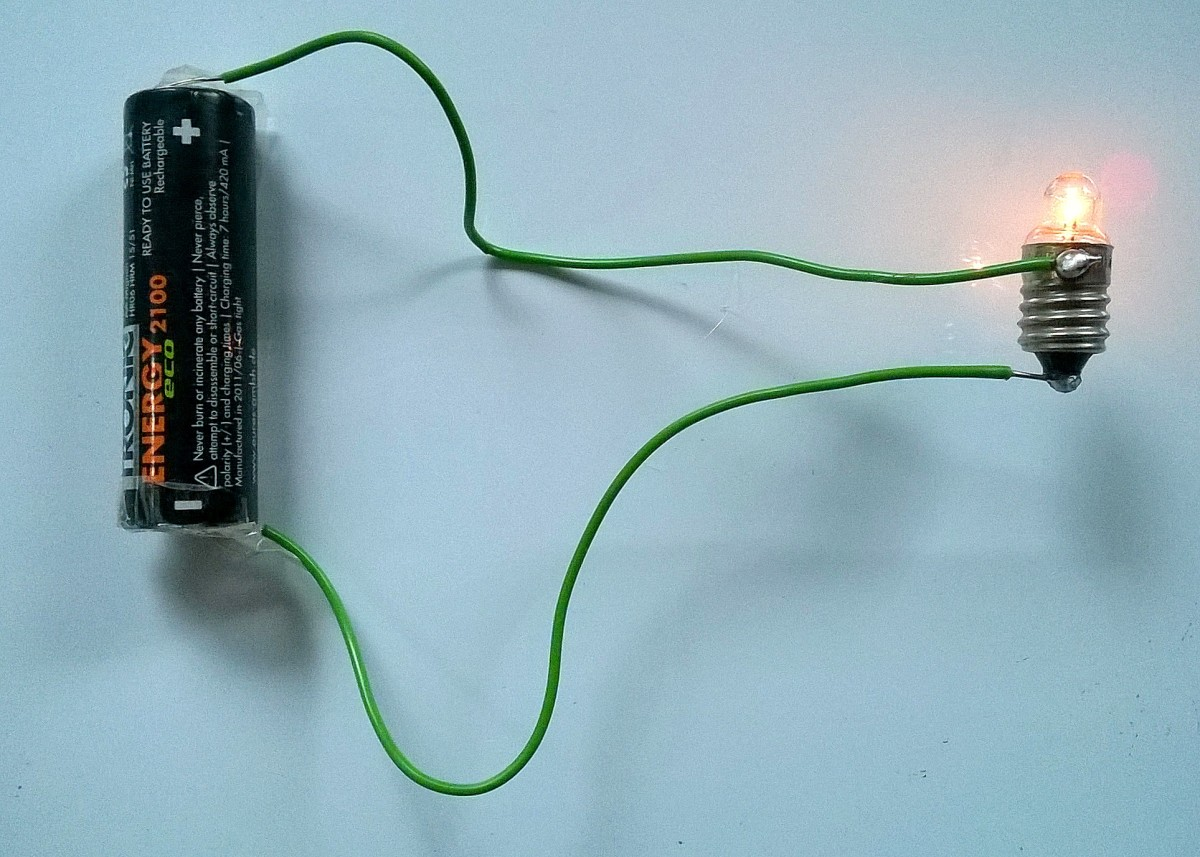In this example of a simple circuit, an AA cell forces current through the wires and lights up a bulb© Eugene Brennan

We can represent this circuit in a simple manner using a schematic or circuit diagram. Looking at the schematic below, a voltage source V will force a current I around the circuit through the load (the bulb in this case) whose resistance is R.
The load R could be an electrical appliance such as a heater, bulb, LED, motor or component in an electronic circuit. The lines joining the source to the resistance would be the connecting wires inside an appliance or power flex, or tracks on a printed circuit board.

Ohm's Law
The current I is given by the equation:

I = V/R

We'll cover Ohm's Law in more detail later and see some examples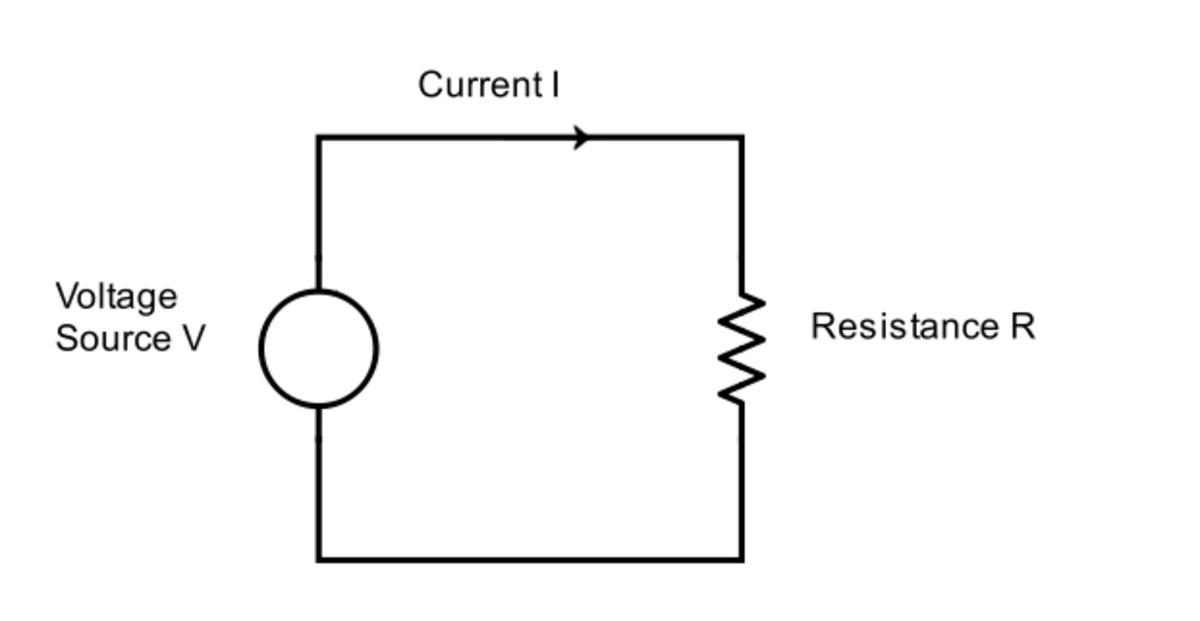A schematic of of a simple circuit. The voltage source V causes current I to flow around in a loop through the resistance or load R.© Eugene Brennan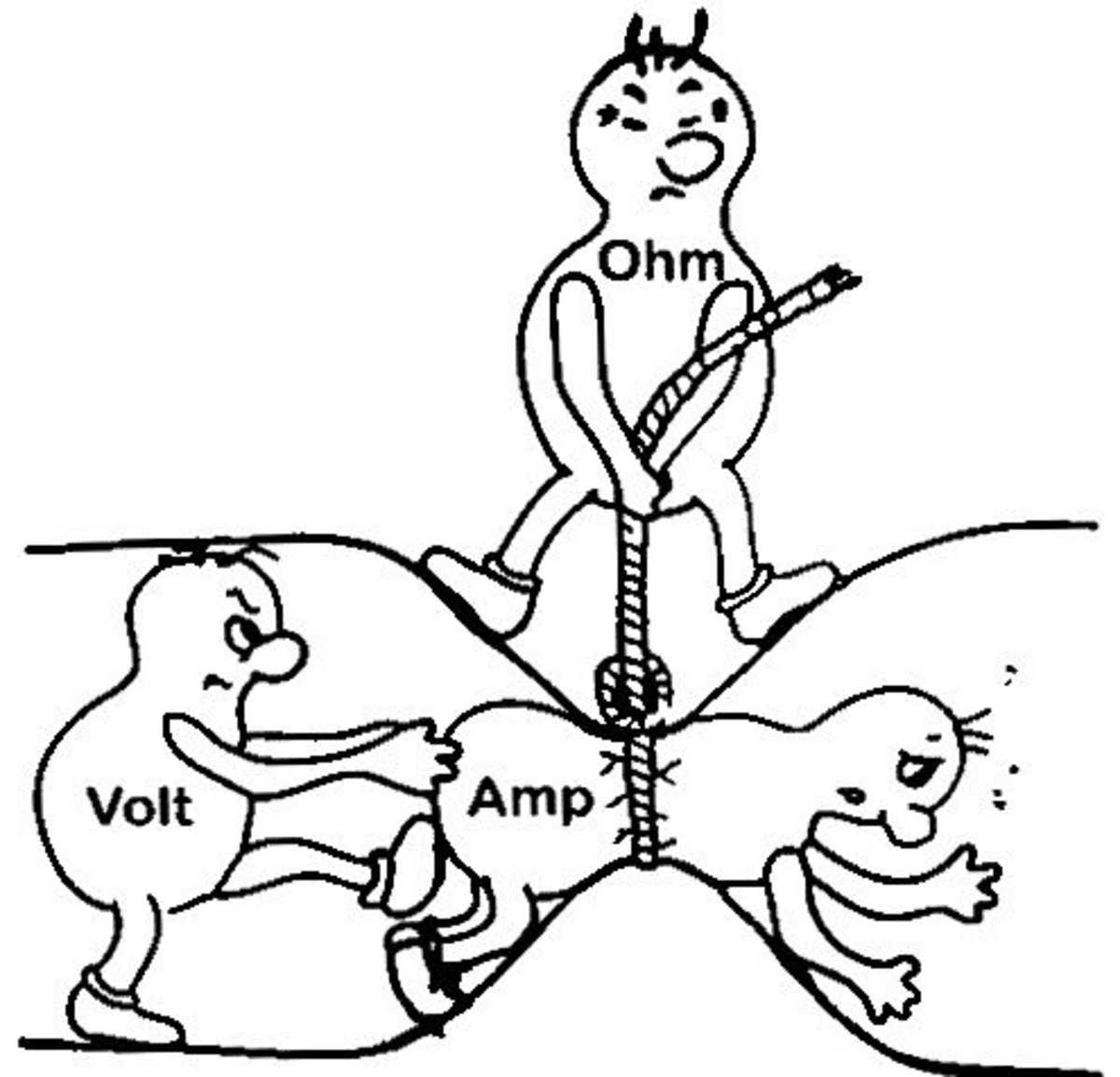Voltage (measured in volts) forces current (measured in amps) through the resistance of a circuit (measured in ohms).

## Current Direction in a Circuit

Conventionally we think of current flowing out the positive terminal of a source such as a battery. However current is a flow of sub-atomic particles called electrons which are negatively charged, so current actually flows the other way, from the negative terminal of the battery.

## What are Examples of Voltage Sources?

• Battery
• Mains voltage at a socket outlet
• Alternator or DC generator (dynamo)
• Solar cell
• Thermopile
• Laboratory power supply

## The Water Pipe Analogy for Explaining Electricity

Voltage and current are like water pressure and water flow rate respectively, and reference is often made to pumps and water pipes as an analogy to explain electrical circuitry.

## What's the Difference Between Volts and Amps?

Like any discipline, electrical engineering has jargon or specialized terminology.

• ### What Are Volts?

Voltage is the pressure in a circuit and measured in volts. Think of a pump in a water pipe. The greater the pressure and the force which the pump exerts, the greater will be the flow of water through the pipe. Similarly a voltage source is like a pump and pushes electrons around the circuit. The higher the voltage applied to a circuit, the greater the current which will be forced through it.

• ### What Are Amps?

An electric current is due to the movement of electrons through a conductor and load and is measured in amps. High current means lots of electrons flowing through the circuit. The water analogy is water flow rate in gallons per minute.

• ### What is a Load?

This is the device connected to a voltage source. It could be a motor, bulb, heater, LED, or an electronic resistor.

• ### What Are Ohms?

A load has resistance and this is measured in ohms. Every electrical device or load has resistance. Resistance is like a restriction to the flow of electrons and electricity is dissipated as heat energy in a resistance. For a fixed voltage applied to a load, the higher the resistance, the lower the current. Going back to the water analogy, when you stand on a hose, you increase the resistance and restrict the flow. The only way to restore the flow is by getting the pump to pump harder, and force water through the restriction, i.e. the pump needs to have a higher pressure. Alternatively if you take your foot off the hose, you increase the diameter and lower the resistance and more water can be forced through. In an electrical circuit, if the voltage is increased, more current is forced through the resistance. If the resistance is lowered, more current will flow even if the voltage of the source doesn't change. Even connecting wires in a circuit have resistance, so when high currents need to be carried by a cable, the copper or aluminum cores need to have a sufficiently large cross sectional area (CSA) to avoid overheating.

• ### What Are Watts?

Power is the rate at which energy is consumed by a load and is measured in watts. A kilowatt is 1000 watts, also abbreviated to kW. Low powers are measured in milliwatts (mW) or thousandths of an amp.

• ### What Are KWh or Kilowatt Hours?

Kwh are a measure of energy consumption. KWh are sometimes called units and are what you pay for on your electricity bill. A 1 kilowatt (1000 watt) appliance uses a kilowatt hour of electricity in one hour. Similarly a 500 watt device uses a kilowatt hour of electricity in 2 hours.

• ### What are Hertz?

For an AC supply, the frequency is the number of times per second that the current changes direction, measured in cycles per second or hertz. Electricity is distributed to homes at 50 or 60 hertz.

## What Are Some Commonly Used Voltages ?

Commonly used voltages of devices and systems.

Voltage SourceVoltage

AA or AAA cell

1.5 volts

Mains supply in the home

Nominally 120 or 240 volts

Car battery

12 volts

Truck battery

24 volts

Voltage input to transformer supplying home

Greater than 10 kV (kilo volts)

High voltage transmission lines

Up to 1.2 MV (Mega volts)

## How to Convert Between Volts, Amps and Watts

Now let's examine the quantities which are usually of interest when dealing with appliances, such as volts, amps and watts and how to convert between them. If you look at the casing of an appliance (see photo below) you can usually find a specification label or panel which indicates the voltage supply, frequency, wattage and possibly current. On some appliances e.g. TVs and washing machines, this panel may be mounted at the back of the device.
This is the equation we need to remember. Once we know it, we can rearrange to find the other two.

## Examples of Working Out Watts, Amps and Volts For Appliances

### How to Work Out Watts

Watts = Volts x Amps

e.g. A 120 volt appliance takes 2 amps, what is the power?

Power in watts = 120 x 2 = 240 watts

### How to Work Out Amps

Amps = Watts / Volts

e.g. A 240 volt appliance consumes 480 watts of power, How much current does it draw?

Current in amps = 480 / 240 = 2 amps

### How to Work Out Volts

Volts = Watts / Amps

e.g. A 720 watt appliance draws 3 amps, What voltage is it running on?

Voltage in volts = 720 / 3 = 240 volts

So it's really that simple. Notice I have chosen values in the examples so that everything works out nicely. You only really need to remember the first equation and if you know basic algebra you can rearrange to give the other two equations. However as you can see, you always need to know two of the quantities before you can work out the third quantity. From looking at the Google Analytics statistics and the questions which land people on this webpage, I often see questions asked such as "how many watts are in 480 volts?", which obviously makes no sense!

For high powered appliances, power is often specified in kilowatts ( abbreviated to kW)

1 kilowatt = 1000 watts

## What Is a kWh? — How to Calculate Energy Consumption of Appliances

Power is the rate at which a device uses energy. So for instance an air conditioning unit, shower or kitchen range/cooker uses electrical energy much faster than a light bulb

### Energy used = Power x Time

So to figure out the energy usage of an appliance, you multiply its power rating by the time period for which it is running. The standard unit of energy is the joule or calorie, but generally energy used in the home is measured in kWh, also known as "units". To work out the number of kwh, you divide the power in watts by 1000 to convert to kilowatt (kW) and then multiply by time in hours to give kWh. So:

### kWh = Watts / 1000 x time in hours

Kilowatt hours, kWh or units are what you pay for on your bill. Your electricity meter counts and displays the number of units used by all the appliances and lighting in your home.

e.g. A 2500 watt drier runs for 3 hours a day, how many kWh does it consume and if electricity costs 12c per unit, what is the cost of running it?

kWh = watts/1000 x time = 2500 / 1000 x 3 = 7.5 kWh or units

Cost = 7.5 x 12c = 90 cents

Some appliances don't run continuously. Examples are devices controlled by a thermostat such as refrigerators, freezers, ovens in cookers and air conditioning systems. The time for which the appliance is powered on and consuming power is called the duty cycle and it is often quoted as a percentage. So for instance a fridge which stays on half of the time has a duty cycle of 50%.

## Labels on Appliances Showing Volts, Current and Power Rating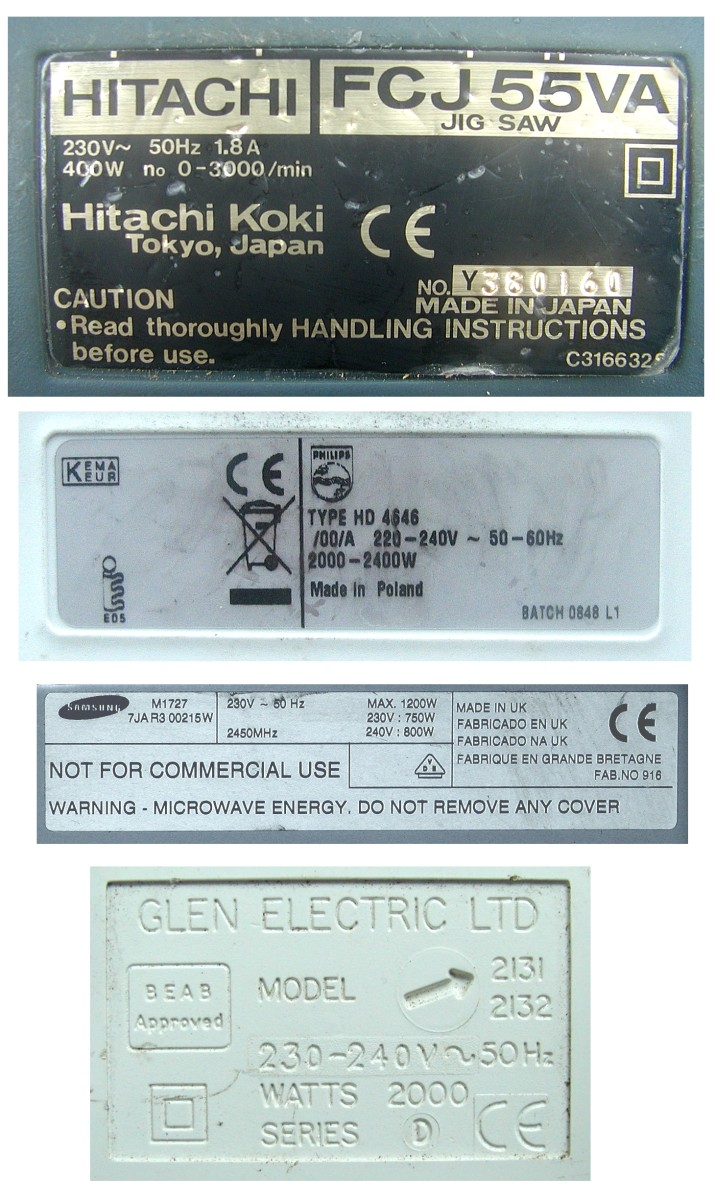Typical electrical appliance labels/panels indicating voltage and current rating, power rating and frequency in hertz.© Eugene Brennan

## Calculating Power Consumption of Electric Appliances

See my guide What is the Cost of Running Electrical Appliances? for a comprehensive list of home appliances, their power consumption and how much it costs to run them per hour.

## How to Convert Horsepower to Watts

Horsepower is a measure of....you guessed it!..... power!

Just as an engine's mechanical output can be measured in horsepower, so can the power of an electric motor.

### 1 horsepower = 746 watts

E.g. A fractional horsepower motor in a washing machine is rated at 1/2 horsepower

So the power output of the motor = 746 watts x 0.5 = 373 watts

A motor is not 100% efficient, in other words not all the electrical power input is converted into mechanical power at the output shaft, some being wasted as heat in the windings.

## Recommended Books

Introductory Circuit Analysis by Robert L Boylestad covers the basics of electricity and also more advanced topics such as AC theory, magnetic circuits, electrostatics and electronic simulation using SPICE based software. It's well illustrated and suitable for high school students and also first and second year electric or electronic engineering students. This hardcover 10th edition is available from Amazon with a "good - used" rating. Later editions are also available.

## Ohms's Law and Electrical Resistance

In the circuit above, a voltage V pushes a current I around the circuit and through the load. As you may remember, this could be a device such as a bulb, electrical heater, motor, LED or other electrical appliance. The load resists the flow of current and the magnitude of its resistance is R ohms.

So

or

### R = V / I

This is known as Ohm's law and basically says that the current is proportional to the voltage and inversely proportional to the resistance (As resistance increases, current decreases and vice versa) Remember the resistance measured in ohms is just a measure of how the load or appliance in the circuit "resists" the flow of current. In electronic circuits and some electrical appliances, components called resistors have precise values of resistance so that they can be used to control the value of current flowing in a circuit.

An example:

The resistance in a circuit is 100 ohms, a voltage of 120 volts is applied, what is the current?

Current = 120 / 100 = 1.2 amps

## How to Work Out Power in a Circuit Knowing the Resistance and Voltage or Current

Remember watts = volts x amps? An alternative way to work out power is from the resistance in ohms:

So if I is the current in amps, V is the voltage, R is the resistance in ohms and P is the power in watts,

Then:

I = V / R from Ohm's law

But also watts = volts x amps, i.e. P = VI

So substituting the expression I =V/R into P = VI gives:

similarly

### P = VI = (IR)I = I2R

It's unlikely when dealing with appliances in the home to need to use the last two equations. However here is an example.

A 240 volt supply is connected to a load of 100 ohms. What is the power consumption of the load?

Power = V2/ R = (240)² / 100 = 576 watts

## Summary of Equations for an Electric Circuit

 Voltage V = IR Current I = V/R Resistance R = V/I Power P = IV Current I = P/V Voltage V = P/I Power P = V²/R Power P = I²R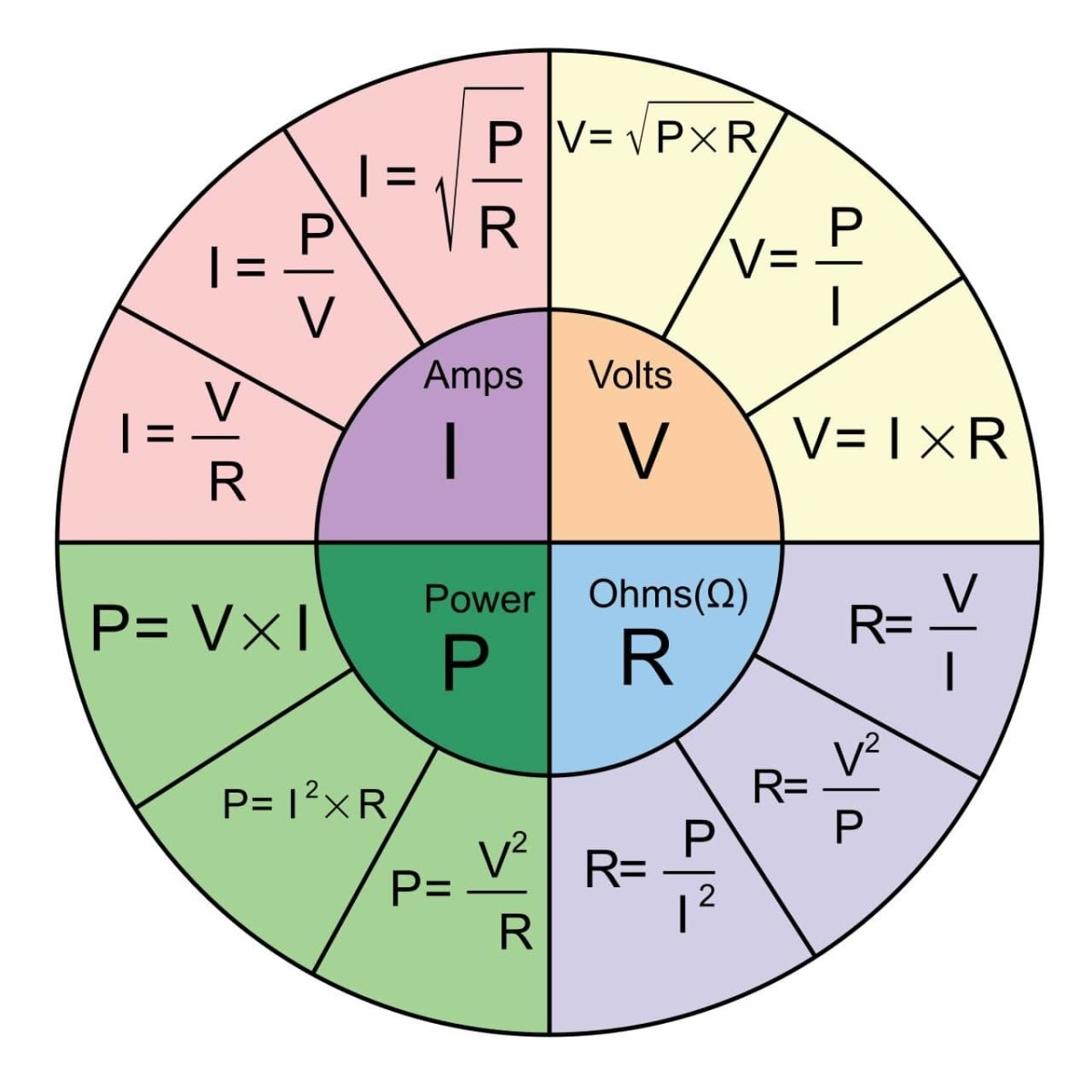Summary of equations for an electrical circuit. P is power, E is the voltage, I is the current and R is the resistanceMatt Rider CC BY SA 3.0 Unported via Wiimedia Commons (changed "E" to "V")

## What is Resistivity and How Does it Affect Resistance?

### What are Electrical Conductors?

A conductor is a physical medium which carries an electric current. This could be a power cable, prongs on a plug, a liquid such as water, battery acid or ionized gas in a discharge lamp (e.g. fluorescent or sodium lamp).

In the case of a solid conductor such as copper wire, the electrical resistance is proportional to the length of the conductor and inversely proportional to its cross-sectional area. In effect this means that the longer a piece of wire, the higher its resistance. Similarly the greater the diameter of the wire, the lower its resistance. This has implications for conductors used in appliances and power transmission. For example, the gauge of wire used in an extension lead is important, if the wire is too thin, the resistance will be high and the cable can overheat. If a power cable is very long, its resistance may be too high if not properly rated, resulting in an unacceptable voltage drop at the end of the cable (because of the resistance).

For a conductor with cross sectional area A and length l, the resistance R can be calculated using the equation:

### R = ρl / A

ρ (Greek letter "rho") is a constant known as the resistivity and is a measure of how good the material is at conducting electricity. The lower the resistivity of a material, the lower will be the resistance of the conductor.

Copper has the lowest resistivity of most common materials and this is why it is widely used in the manufacture of cables. Silver has a lower resistivity than copper, but it is much more expensive. Aluminium is generally used for overhead cables and although it has a higher resistivity than copper, it is lighter. Gold has a resistivity about 1.5 times that of copper, however it is unreactive and doesn't oxidize (tarnish). A tarnish coating on a conductor increases contact resistance, so this is why gold is often used as a coating on audio / video connectors. Gold is also used for the miniature connecting wires in integrated circuits.
Insulators are conductors with very high and for all practical purposes infinite resistance.

## Resistivities of Various Materials

Materials with increasing resistivity.

MaterialResistivity

Silver

1.59×10−8 Ωm

Copper

1.68×10−8 Ωm

Gold

2.44×10−8 Ωm

Aluminium

2.82×10−8

Iron

9.71×10−8 Ωm

Platinum

1.06×10−7 Ωm

Nichrome (used in heating elements)

1.10×10−6 Ωm

Glass

1.00×1011 to 1.00×1015 Ωm

Hard Rubber

1.00×1013 Ωm

## What is an Insulator?

An electrical insulator is a material which has a very high resistance because there are no free electrons to carry current. For all practical purposes an insulator can be considered to have infinite resistance. Because resistance is infinite (infinity is represented by the symbol ∞), then current through an insulator is:

Current = Voltage / resistance = voltage / ∞ = 0

Insulators are used to prevent current flow between two electrical points with differing voltage e.g. insulation on the individual cores of a power cable, the plastic of a power plug or glass/ceramic insulators on power lines. They also prevent high voltage from causing electric shock.

## What are Insulators Made Of?

Typical insulating material used for electrical purposes are:

• Plastic
• Ceramic
• Glass
• Glass epoxy (used for PCBs)
• Bakelite (an older style thermosetting plastic)
• Mica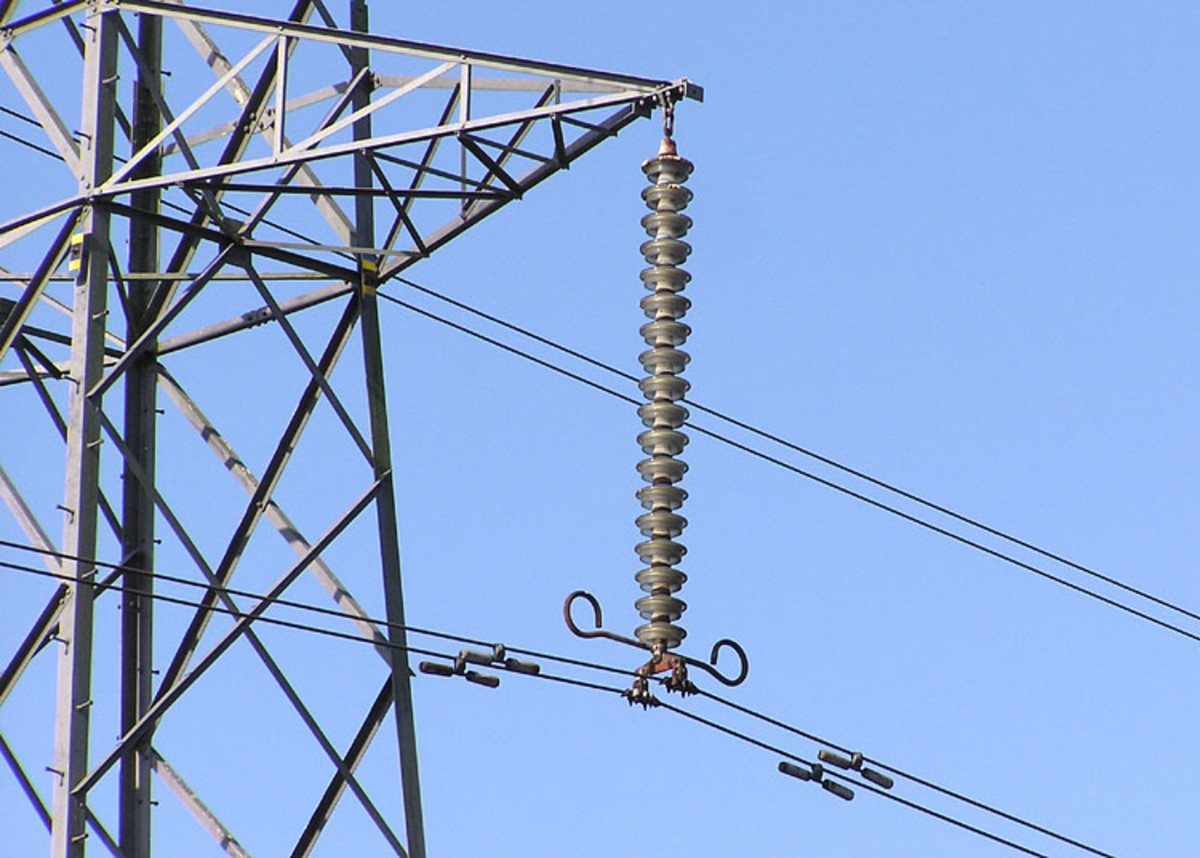Detail of the insulator string (the vertical string of discs) on a 275,000 volt electricity pylon near Thornbury, South Gloucestershire, England.Adrian Pingstone, Public domain image via Wikimedia Commons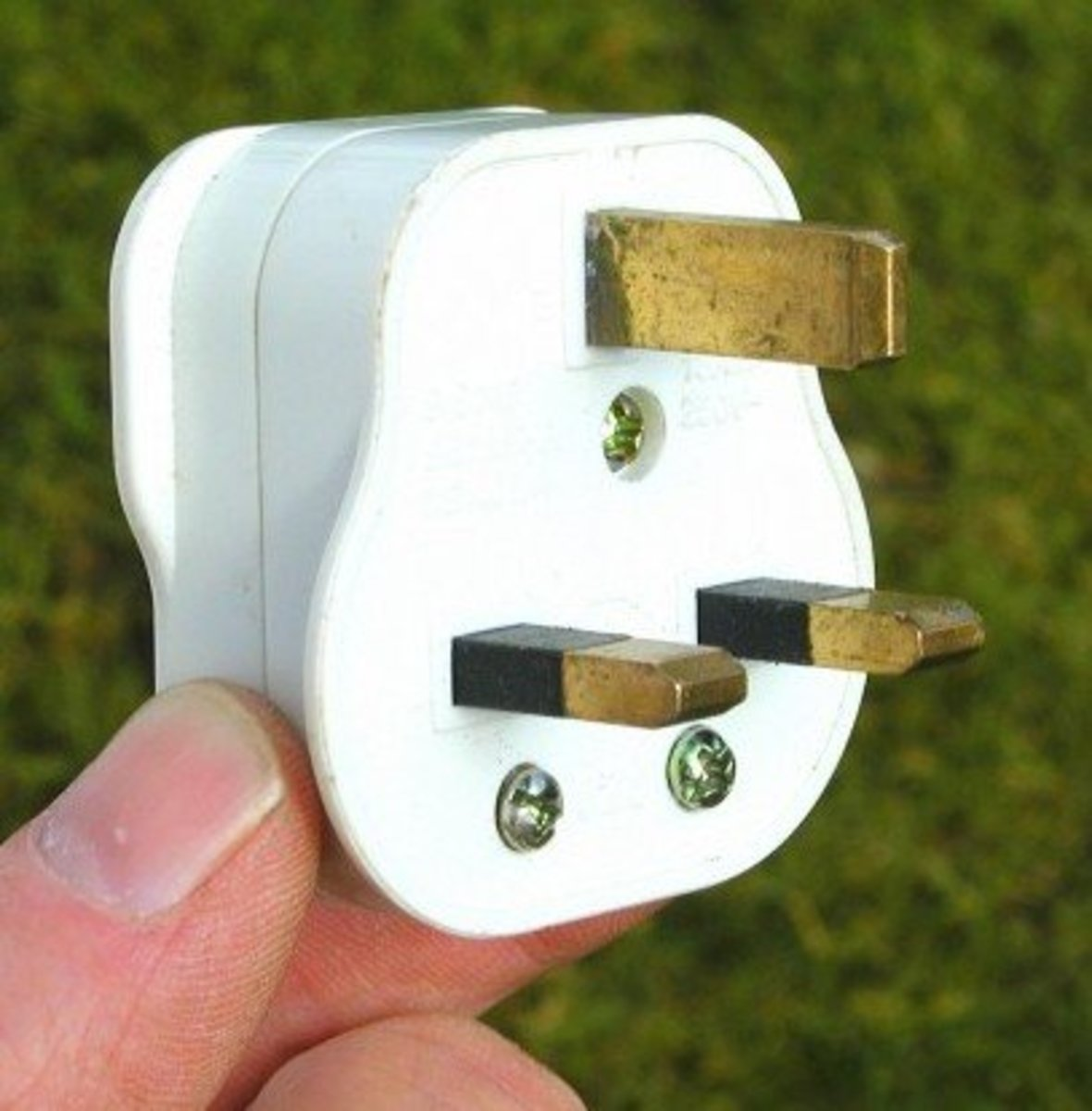The insulating black plastic shrouds on the pins of this plug prevent contact with the pins during insertion/removal.© Eugene Brennan

Since electricity is a flow of electrons, it isn't really made. Instead it is produced or generated when these electrons are moved.

Electricity is produced from:

• Batteries
• DC generators or AC alternators
• Solar cells
• Thermopiles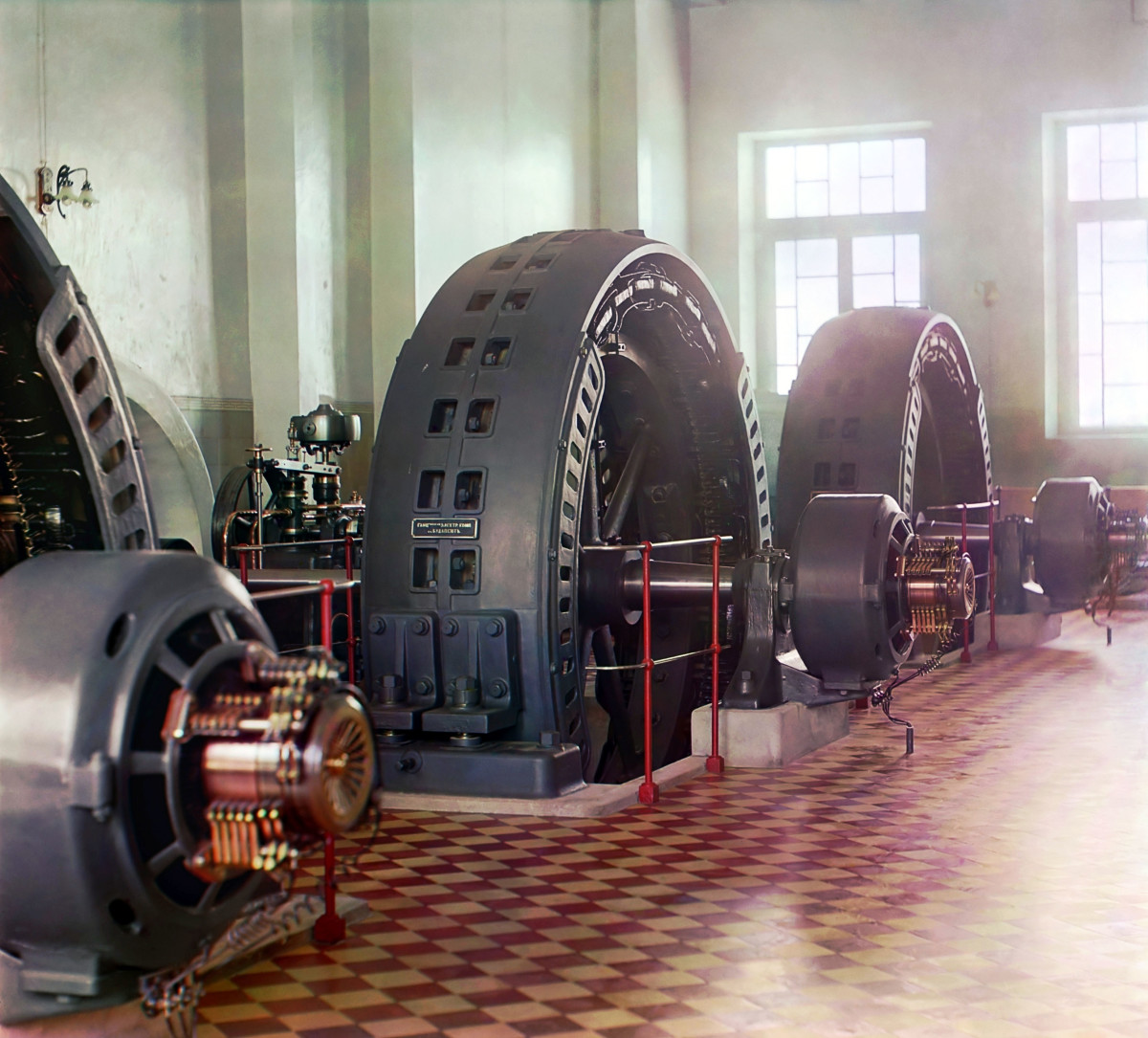Early 20th century (1909) alternators in a hydroelectric power station.Sergei Prokudin-Gorskii - Public domain image via Wikipedia

## What Does a Power Plant Do?

A power station, also known as a power plant generates electricity using alternators or solar cells. There are several types of power plants, thermal, hydroelectric, wind, wave, tide and solar.

### Power stations that use alternators to generate electricity:

Hydroelectric Power Station

In a hydroelectric power station, water flowing through pipes from a dammed lake turns the blades of a turbine attached to the shaft of an alternator. The alternator then generates electricity.

Thermal Power Station

Fossil fuels such as coal, oil, gas and peat or renewable energy crops like willow are burned and the heat is used to boil water and generate steam at high pressure. The steam passes through pipes to a steam turbine and turns it at high speed. Again the steam turbine is connected to the shaft of an alternator, turning it and generating electricity. Nuclear power stations are also thermal using the heat of nuclear fission to boil water and turn it into steam.

Wind Farm

A wind farm uses windmills to generate electricity. Wind turns the blades of the windmill which are connected to a metal shaft. This shaft turns an alternator and this generates electricity. Wind farms can have several hundred windmills spread over hundreds of acres.

Wave and Tide Generation

Wave energy generators use the motion of waves to operate an electric generator. Tidal generators are like undersea windmills and use the flow of water during rising and ebbing tides to turn giant under water "propellers". Like a windmill, the propeller is connected to an alternator that generates electricity.

### Power stations that don't use alternators to produce electricity:

Solar panels don't have any moving parts unlike an alternator

Solar Farm

Solar panels are large flat panels made of special semiconductor material. When sunshine lands on the panels, they produce an electric current. The larger the area of the panel, the greater is the electricity produced. Just like wind farms, solar generating farms can be spread over a large area and consist of hundreds of panels. However people can also have solar panels fixed on their roof to generate some of their electricity requirements. Solar panels are becoming more efficient, which means that they can produce useful amounts of electricity even on cloudy days.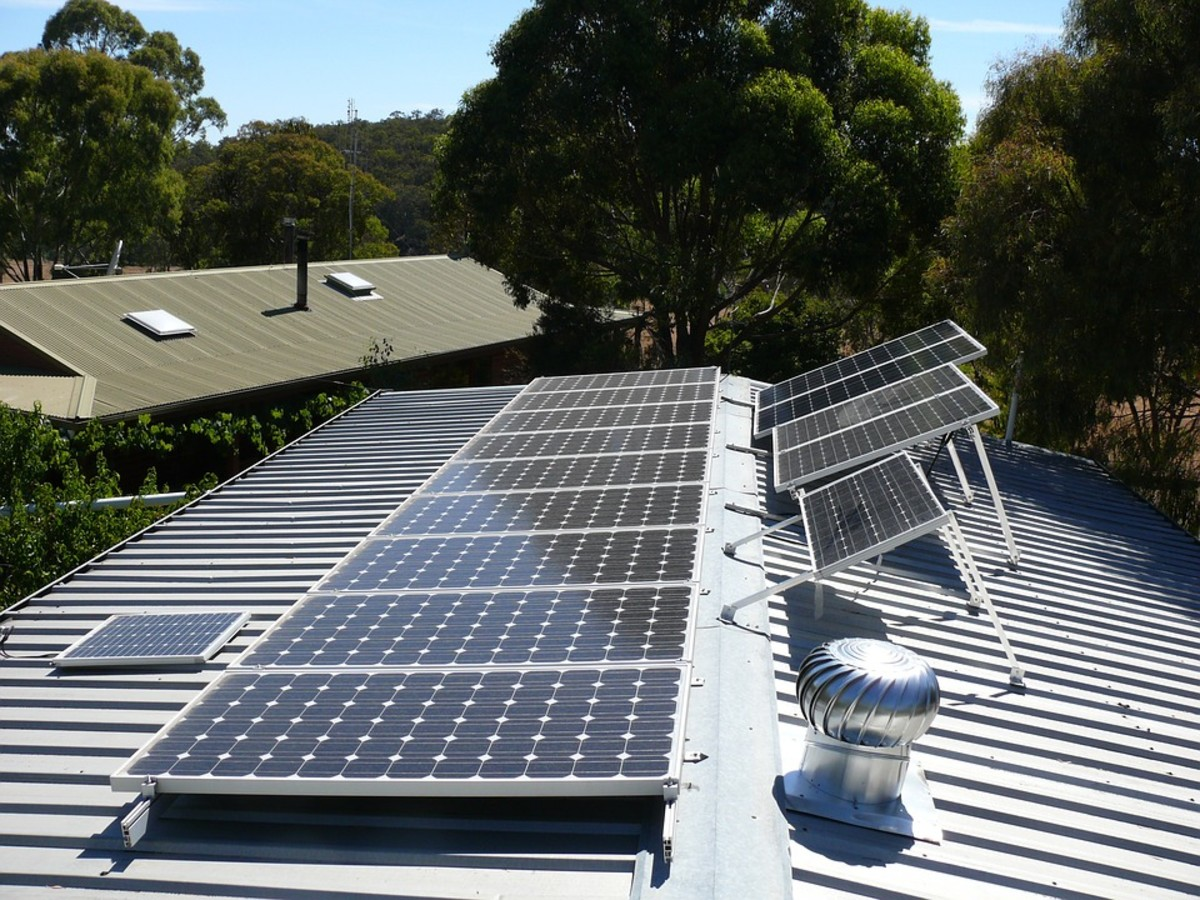Photovolataic solar panels on a roof.RosiePosie, public domain image via Pixabay.com

## What is a Thermopile?

A thermopile is an array of thermocouples connected together, usually in series. A thermocouple works on the principle of the Seebeck Effect to produce electricity. Thermopiles aren't really used to produce energy commercially, but are the only method of generating the electricity required by probes in deep space, far from the Sun. Solar power isn't an option and batteries wouldn't have the capacity to last the mission. So radioisotope thermoelectric generators (RTG) are used consisting of a nuclear source to produce heat and a thermopile to produce electricity.

## What Is the Voltage Supplied to Our Homes?

In general, the voltage supply to your home is nominally 230 or 120 volts. Voltage in the USA is 120 volts, but two "hots" are supplied to homes so that a 240 volt supply is also available between the hots. The higher voltage is used for high powered appliances such as washers, driers, kitchen ranges (cookers) and air conditioning. 120 volts is used for lower power and portable devices. It is also safer because in the event of an electrical shock, less current flows through the body so there is a lesser risk of electrocution.

In countries where 230 volts is standard, generators or step down isolating transformers are used to provide a 110 volt supply for power tools. This is normally mandatory on construction sites. Again the idea of the lower voltage is to lessen the danger of electrocution, if for example a power flex is inadvertently cut, or a tool gets wet.

## What Are Fuses For?

As we will see later, electrical cables, appliances, wires inside appliances, components etc all have resistance. This resistance produces heat when current flows through it. Any electrical conductor can get excessively hot if too much current flows and in the case of wires, this can cause the plastic insulation covering the cable to melt or even catch fire. So fuses are used in series with a cable or appliance to limit current flow and make everything safe. Fuses are like a "weak link" in a chain and blow before damage can occur. They have a specified rating and this is not the current they blow at, but the current they will carry without blowing. Once current exceeds the rating of the fuse, the fuse will blow. The length of time it takes for the fuse to blow is proportional to the current. So minor overloads can result in a fuse blowing in minutes, but if there is a large current or short circuit scenario, the fuse will blow in seconds or milli-seconds.

### Breaking Capacity of Fuses

Fuses have a max current they can carry without the encapsulation of the fuse rupturing. So fuses on the secondary of domestic power supplies in TVs, battery chargers and other electronic appliances are often glass types because the supply will only source a relatively small amount of energy if there is a fault. Ceramic types are used to resist the heat and shock that occurs when the inrush current can be perhaps hundred or thousands of amps, potentially feeding a huge amount of instantaneous power. If a short circuit occurs in an appliance, it's quite possible that the utility transformer in your street can feed current of this magnitude into the short. So for example the BS1362 fuse in a UK style plug has a ceramic body. Blown fuses should always be replaced by the same type, ceramic if necessary, to avoid a fire occurring.

### Fuse Types

In general, fuses are fast blow (F) and time lag (T). Time lag types are often used for power supplies in electronic equipment because the capacitors take a surge of current as they charge up, which would blow a fast acting fuse.

## How Many Amps Are Supplied to Our Homes?

Typically for a 230 volt supply to a home, the main fuse rating is 80 to 100 amps at the consumer unit. So this is the maximum current that will flow before the fuse blows. At 80 A and 230 volts, this allows a power draw of 230 x 80 = 18.4 kW.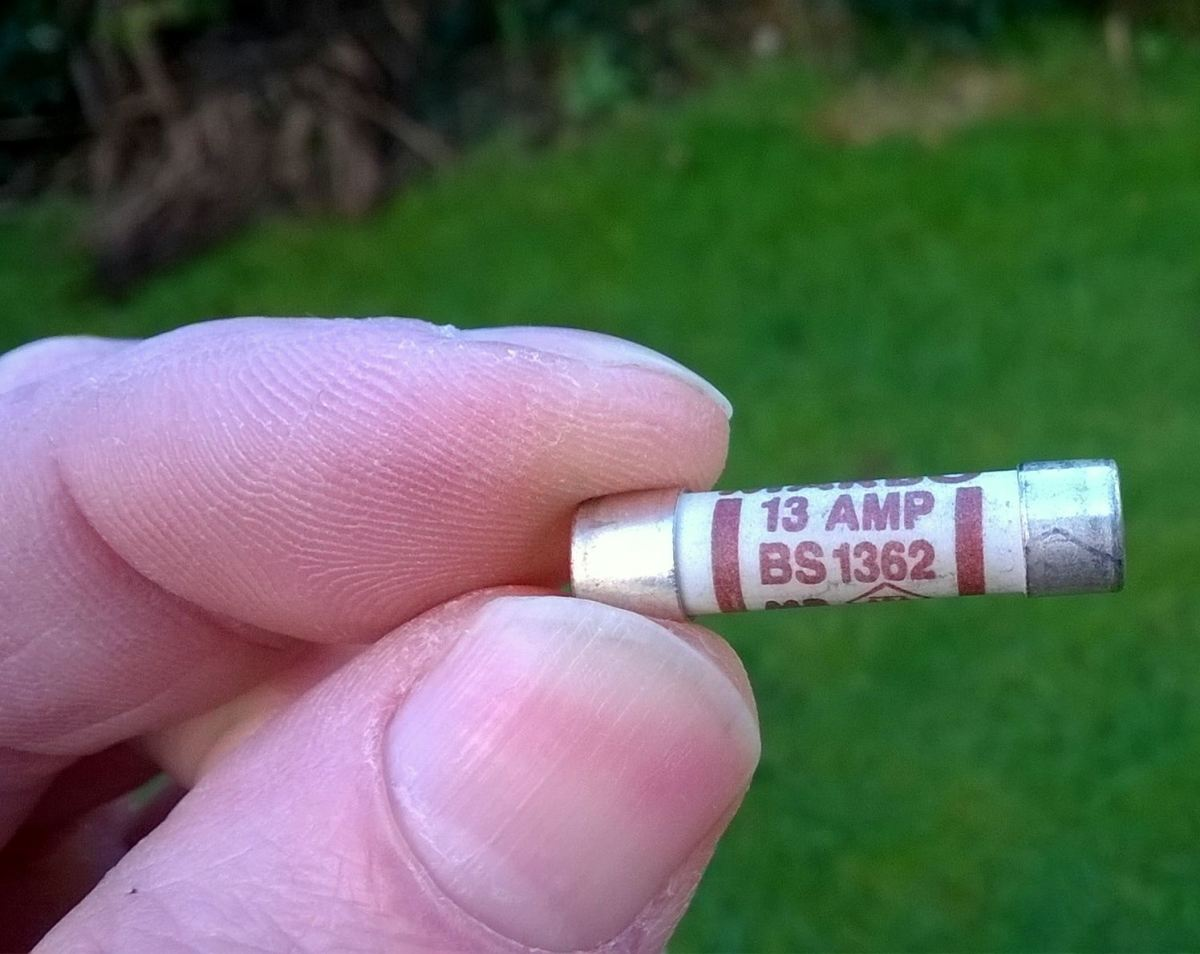A High Breaking Capacity (HBC) BS1362 fuse, used as standard in a UK style plug.© Eugene Brennan

## How Do You Check Voltage With a Multimeter?

A multimeter is an instrument which can measure voltage, current, resistance and possibly additional parameters. You can also use it to check continuity of cables and check fuses. If you don't know how to use one, read my guide How to Use a Digital Multimeter (DMM) to Measure Voltage, Current, and Resistance. Multimeters normally have a continuity range also, and this comes in useful for checking breaks in cables, fuses and loose connections.

## Digital Multimeters From Amazon

Fluke, who are a leading manufacturer of digital instrumentation, recommend the Fluke 113 model for general purpose use in the home or for car maintenance. This is an excellent meter and can measure AC and DC volts, resistance, check continuity and diodes. The meter is auto-ranging, so ranges don't have to be set. It is also a true-RMS meter. If you also need to measure AC and DC current, the Fluke 106 is a suitable choice.

## How to Test a Live Wire?

For this it's best to stay safe and use a non-contact volt tester or phase tester screwdriver. These will indicate if voltage is e.g > 100 volts. A multimeter can only measure the voltage between live and neutral or live and earth (ground) if these conductors/terminals are accessible, which may not always be the case.

A Fluke "VoltAlert™" non-contact detector is a standard tool in any electrician's tool kit, but useful for homeowners also. I use one of these for identifying which conductor is live whenever I'm doing any home maintenance. Unlike a neon screwdriver (phase tester), you can use one of these in situations when live parts/wires are shrouded or covered with insulation and you can't make contact with wires. It also comes in useful for checking whether there's a break in a power flex and where the break occurs.

Note: It's always a good idea to use a neon tester to double check that power is definitely off when doing any electrical maintenance.

## What Is an Electricity Usage Monitor?

An electricity usage monitor or tracker tells you everything you want to know about your appliance behavior. The parameters are displayed on an LCD and include voltage, current, power consumption, kwh used, cost of running and run time of appliance. The latter is useful for troubleshooting fridges, freezers, air conditioners etc which are controlled by a thermostat and switch on and off. A failed thermostat or waterlogged insulation can cause an appliance to run constantly, so this problem can be identified.

## Electrical Energy Consumption

What happens when an appliance is powered from electricity? Scientists tell us that energy cannot be destroyed, it just changes from one form to another. This process happens all the time - on Earth and throughout the Universe. For instance a rock on the edge of a cliff has potential energy, because of its altitude above the ground. If it falls over the edge of the cliff, it starts to pick up velocity, i.e. gains kinetic energy (motion energy) while losing potential energy. When it hits the ground, this energy is dissipated as heat (think of the heat produced by an asteroid impact). Similarly when an appliance is plugged in, the electricity doesn't get wasted or "consumed", in the sense of being destroyed, it simply changes form. So in the case of a lamp, it ends up as light energy or as heat energy when a heater is used. Electrical energy can also be converted to sound in a loudspeaker or electromagnetic radiation (microwave oven or radio transmitter), all forms of energy. Electrical energy can also be converted to kinetic energy in an electric motor or to potential energy when an elevator is raised in a building.

Power is a measure of the rate at which energy is used. So for instance a 1000 watt heater or high powered hvac air conditioning system uses energy at a higher rate than a 60 watt light bulb.

## How is Electricity Converted to Other Forms of Energy?

Energy TypeExample

Light

Incandescent light bulb, LED, Fluorescent lamp

Heat

Electric heater, Incandescent light bulb

Sound

Loudspeaker, Thunder

Kinetic

Motor spinning a shaft or driving a vehicle

Potential

Winch or lift raising a load, Electromagnet tensioning a spring

Pressure

Air compressor

Chemical

Battery

## What is a Superconductor?

When certain materials are subjected to very low temperatures, their resistance falls to zero.

Since V = IR, if R is zero, then V becomes 0 even if I is non zero

The consequences of this are that a current can flow even if the voltage source is removed. Because resistance is zero, and no heat is dissipated, huge currents can be carried by thin cables. Superconductors are used for example in MRI machines to carry the high currents required by powerful magnets.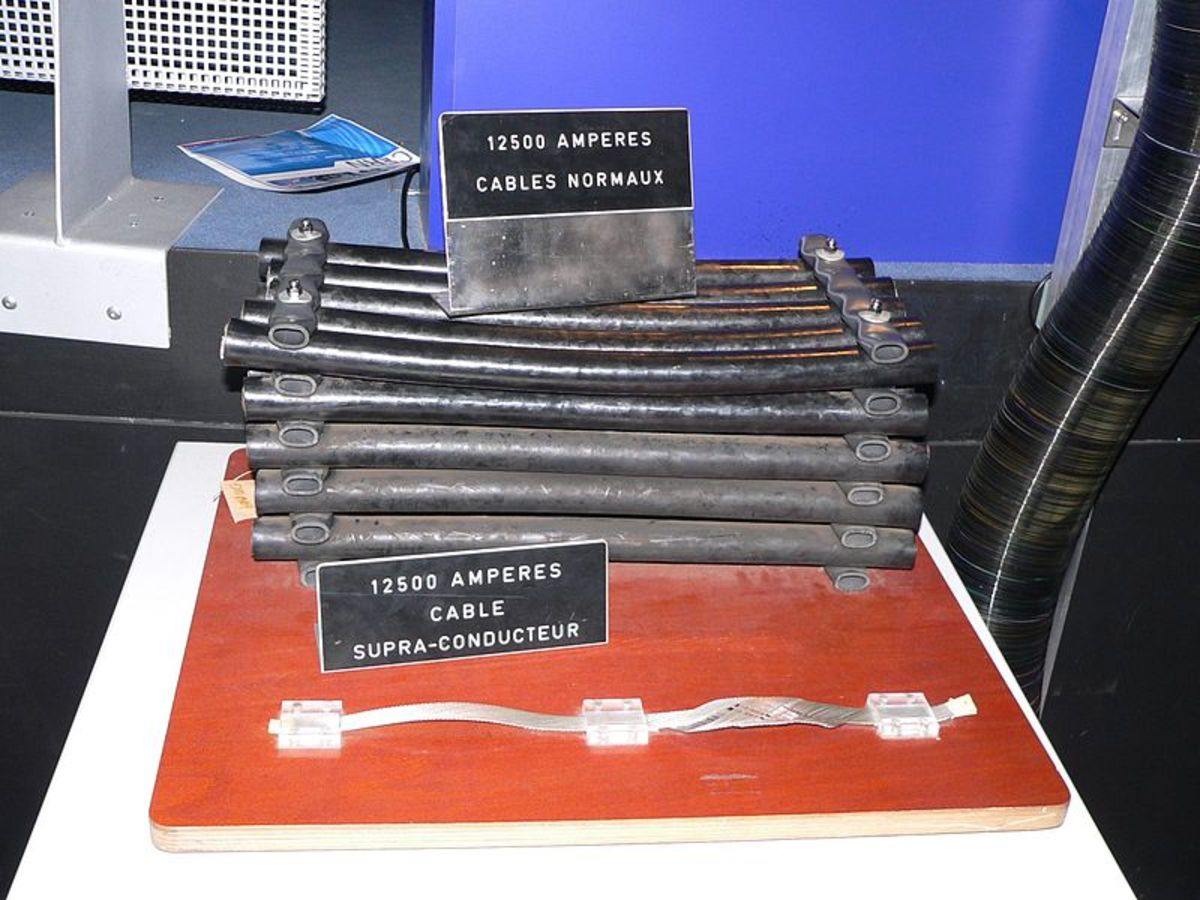Superconducting cables.Rama, CC BY SA 2.0 2.0 France via Wikimedia Commons

## What Are AC and DC?

The current produced by a power source can take one of two forms, AC or DC. The power source could be a battery, electrical generator, power transmitted along service cables to your home or the output of a signal generator, a device used in laboratories or by test personnel when testing or designing electronic systems.

### DC

This stands for direct current so the current provided by the source only flows one way. A DC source will have a nominal value voltage level and this voltage will fall as the source is loaded and outputs more current. This drop is due to inherent internal resistance within the source. The resistance is not due to an actual resistor, but can be modelled as such, and is composed of actual resistance of conductors, electronic components, electrolyte in batteries etc.

Examples of DC sources are batteries, DC generators known as dynamos, solar cells and thermocouples.

### AC

This stands for "alternating current" and means that the current "alternates" or changes direction. So current flows one way, reaches a peak, falls to zero, changes direction, reaches a peak and then falls back to zero again before the whole cycle is repeated. The number of times this cycle happens per second is called the frequency. In the U.S. the frequency is 60 Hertz (Hz) or cycles per second. In other countries it is 50 Hz. The electricity supply in your home is AC.

The advantage of AC is the ease by which it can be transformed from one voltage level to another by a device known as a transformer.

AC sources include the electrical supply to your home, generators in power stations, transformers, DC to AC inverters (allowing you to power appliances from the cigarette lighter in your car), signal generators and variable frequency drives for controlling the speed of motors. The alternator in a vehicle generates electricity as AC before it is rectified and converted to DC. New generation brushless, cordless drills convert the DC voltage of the battery to AC for driving the motor.

### Reducing Costs of Transmitting Electricity Over the Grid

Because AC can so easily be transformed from one voltage to another, it is more advantageous for power transmission over the electricity grid. Generators in power stations output a relatively low voltage, typically 10,000 volts. Transformers can then step this up to a higher voltage, 200,000, 400,000 volts or higher for transmission through the country. A step up transformer, converts the input power to a higher voltage, lower current output. Now this decrease in current is the desired effect for two reasons. Firstly, voltage drop is reduced in the transmission lines because of the lower current flowing through the resistance of cables (since V = IR). Secondly, reducing current reduces power loss as current flows through the resistance of the distribution cables (remember power = I2R in the equations above?). Power is wasted as heat in transmission cables, which obviously isn't wanted. If current is halved, power loss becomes a quarter of what it was previously (because of the squared term in the equation for power), If current is made 10 times smaller, power loss is 1% of what it was, and so on.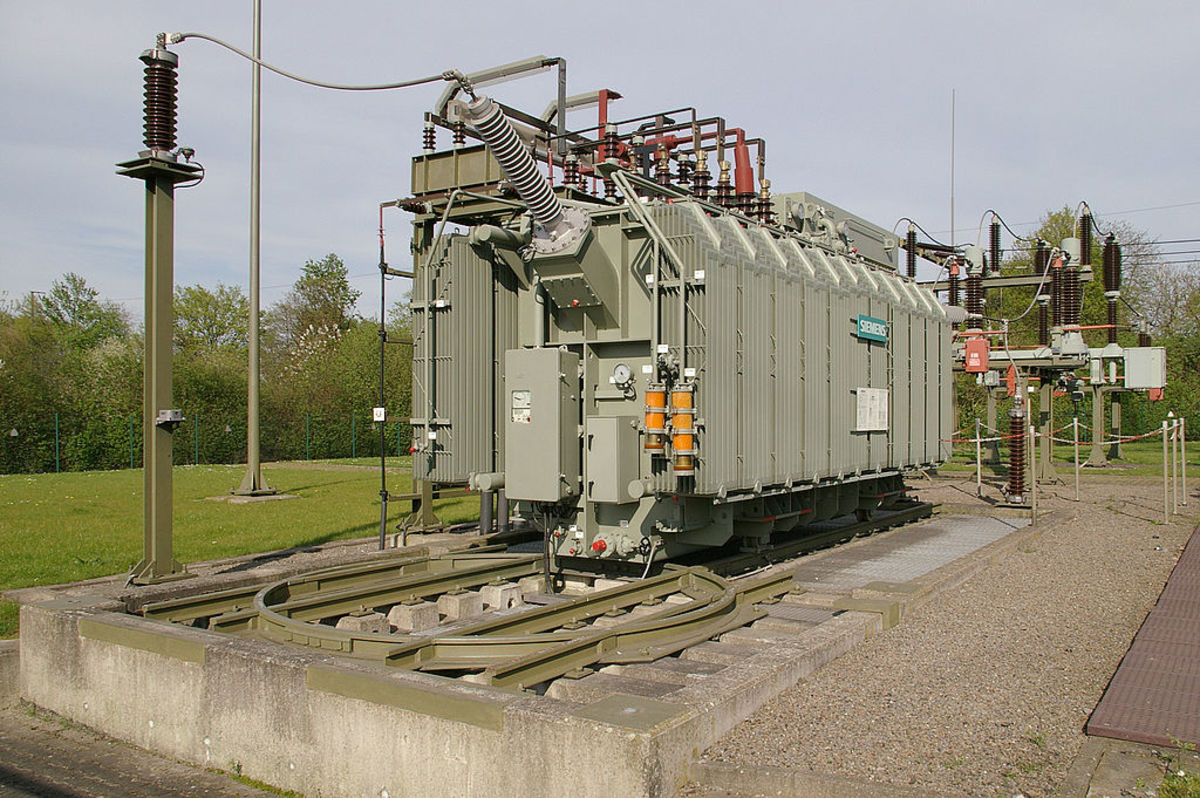Transformer in an electrical sub-station. The function of a transformer is to either increase or decrease voltage.Image: Rainer Knäpper, Free Art License via Wikimedia Commons

## What is Three-Phase Voltage?

Very long distance transmission lines may use DC to reduce losses, however power is normally distributed nationwide using a 3 phase system. Phase voltages have a sinusoidal waveform and each of the phases is separated by 120 degrees. So in the graph below, phase 1 is a sine wave, phase 2 lags by 120 degrees and phase 3 lags by 240 degrees (or leads by 120 degrees). Only 3 wires are needed to transmit power because it turns out that no current flows in the neutral (for a balanced load). The transformer supplying your home, has 3 phase lines as input and the output is a star source so it provides 3 phase lines plus neutral. In countries such as the UK, homes are fed by one of the phases plus a neutral. In the US, one of the phases is split to provide the two 'hot' legs of the supply.

### Why Is 3 Phase Used?

• More power can be transmitted using just 1.5 times the number of wires
• Motors powered by 3 phase are smaller than a similar single phase motor of the same power
• Evening of output torque smooths operation and results in less vibration of motors powered by 3 phase
• Neutral conductor can be reduced in size because of lower current flow
• Neutral is unnecessary for transmitting power between substations and transformers

Note: A "phase" is a single winding in an alternator, winding in a transformer, coil in a motor or resistive load. The phase voltage is the voltage across a phase.

### 3 Phase Formulas

If VP is the phase voltage

and VL is the line voltage between each phase

VP = VL

IP = IL / √3

VP = VL / √3

IP = IL

For both star and delta balanced loads with a power factor of 1:

Power = √3VLIL

### Delta Star Transformer

A Delta-star (also known as delta - wye or delta Y) transformer is often used for producing a 3 phase, or single phase and neutral supply to homes and industry. The incoming supply is typically 11kv and output phase voltage is 230 volts (in countries which use this voltage)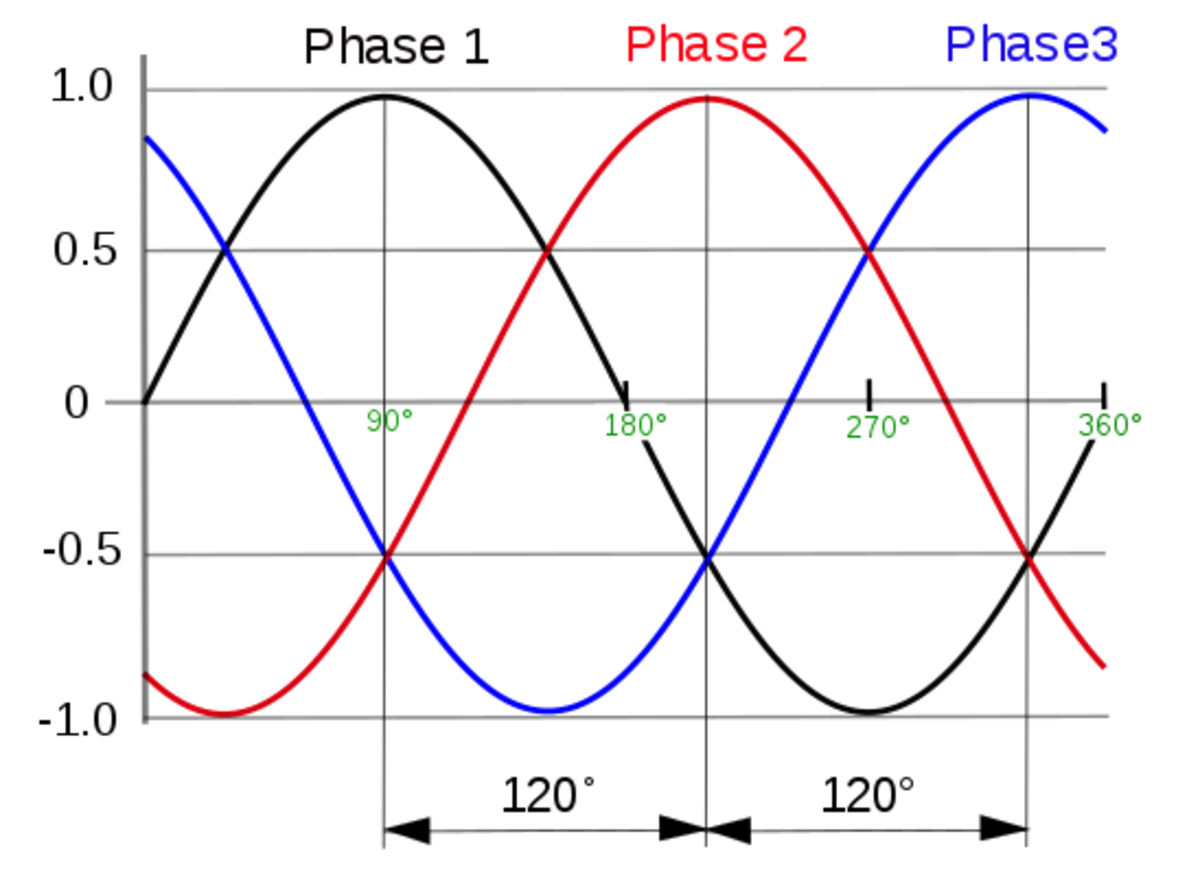3 Phase voltages. Each phase is sinusoidal with a phase difference of 120 degrees.J JMesserly modification of original svg by User:SiriusA, Public Domain image via Wikimedia Commons

## What Are Other Effects When a Current Flows?

As mentioned above, when current flows through the resistance of a load, it gets hot. This is sometimes the desired effect, e.g. an electrical heater. However it is an unwanted effect in lamps, because the desired function of the device is to convert electricity to light, and not produce heat as a byproduct. Excessive current in power cables during an overload can potentially cause a fire if protective devices such as fuses or MCBs (Miniature Circuit Breakers) aren't included in line with the cable.

### Current flow produces:

• Magnetic fields. This phenomenon is used in a device called a solenoid or electromagnet which is basically like a spool or coil of wire through which a current flows. Electromagnets are used in the old style, non-electronic, door and phone bells, water inlet valves on washing machines, relays (a switch operated by an electromagnet), starter motors on vehicles and in salvage for lifting iron and steel.
• Electric fields. Current flowing through a conductor also produces an electric field. An extreme example of this is the high intensity field produced under a high voltage power line which is sufficient to illuminate a fluorescent tube held in the hand.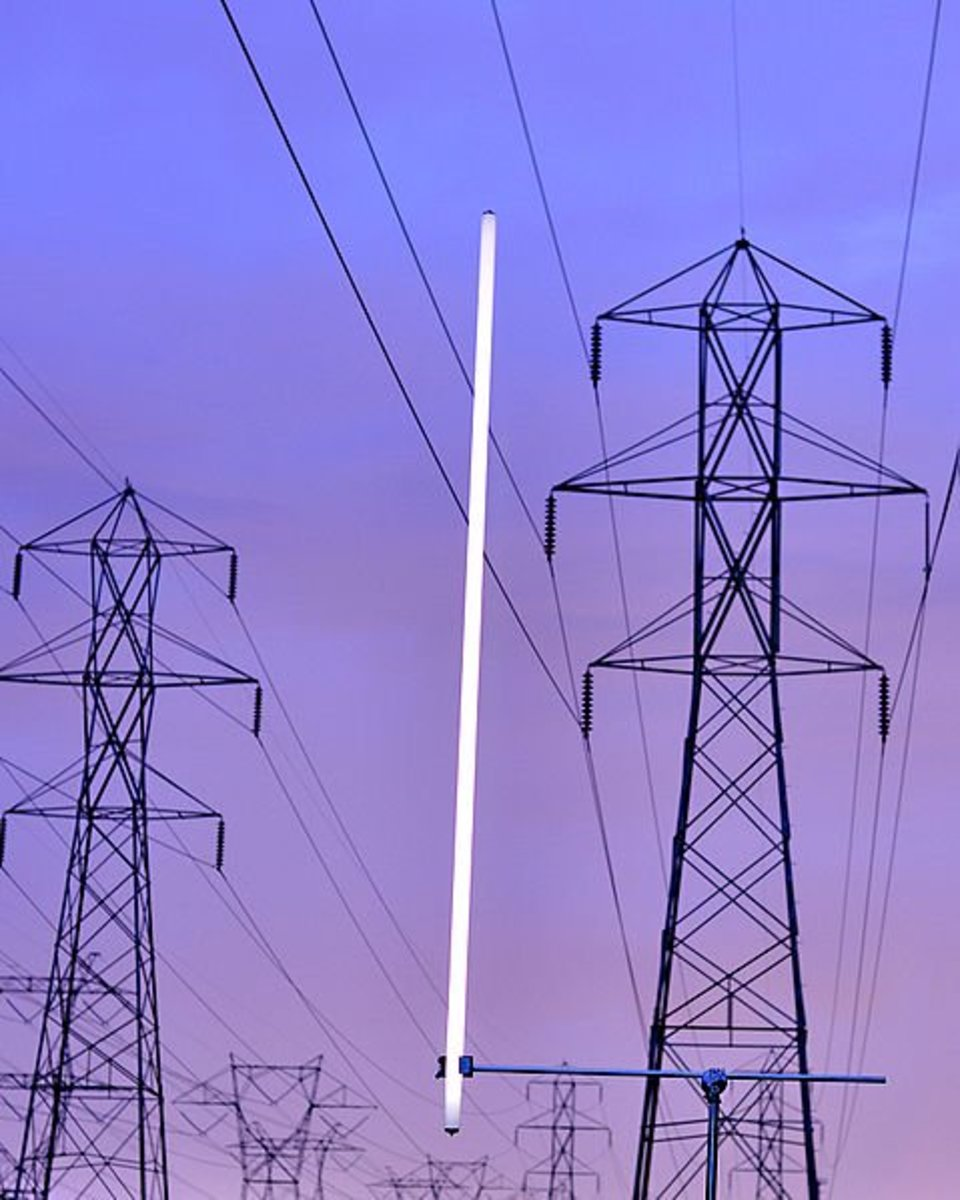The electric field under a high voltage power line is sufficient to produce an electric discharge in a fluorescent tube.Image BaronAlaric GNU_Free_Documentation_License version 1.2 via Wikimedia Commons

## How Do Switches Work and What Are Sparks?

As you've discovered, if resistance is increased in a circuit, current decreases. If you just break the conductor in a circuit and create an air gap, the magnitude of the resistance for all practical purposes is infinite because air is a good insulator and no current will flow. I.e.

Current = Voltage / Resistance = Voltage / = 0

So this is how a switch works. Two contacts, usually made of brass in a domestic switch, are pressed together when the switch is on and closed. When the switch is turned off, the contacts rapidly separate and interrupt current.

### What are Sparks?

Imagine two electrodes or points in a circuit separated by an air gap (e.g. the gap in an automotive spark plug). If voltage is high enough, the air between the two points becomes so stressed by the electric field that it becomes ionized, i.e. atoms have their electrons ripped off. These electrons are then able to traverse the gap, attracted by the positive electrode and in doing so, collide with other gas molecules and release more electrons. Eventually an avalanche of electrons occurs (all of this happening in a split second) and the result is called a spark or spark discharge A spark produces a flash of visible light, heat, UV radiation and sound and it's temperature can be about 5000 deg C, hotter than the surface of the sun. The voltage required to produce a spark is about 3000 volts per mm between rounded electrodes in air. Sparks can be small, e.g. automotive spark plug or gas lighter, or much larger.

An example of a large spark is lightning. When clouds get charged up, voltage becomes so high that a spark jumps from cloud to cloud or cloud to ground. The sound we call thunder is caused by the explosive heating and expansion of air by the electrical discharge.

Sparks occur in an air gap when voltage exceeds the breakdown voltage of the gap. When two electrodes are separated, current tends to continue to flow and heating of the metal electrodes causes material to vaporise and also ionise the air. This results is a continuous spark discharge called an arc which is similar to a spark. If the electrodes are separated sufficiently, the arc won't be sustained and will stop abruptly. Arc welding makes use of an arc between two electrodes to melt metal. Switches must also be designed so that their contacts separate sufficiently apart and quickly enough so that arcs are rapidly quenched and reduce damage to the contacts. In substations, large air gaps or oil filled circuit breakers are necessary to quench the high current arcs which occur when high voltage is switched.

## What is a Voltage Regulator?

A voltage regulator is an electronic device used to keep the voltage output of a power supply at a constant level, independent of current drawn by a load. In general, these devices are implemented as single ICs in a variety of package formats, or as separate modules consisting of several discrete components or integrated circuits. A regulator that reduces voltage is called a buck regulator and one that increases voltage is called a boost regulator.
The output of an unregulated voltage supply will drop as current increases. This is because of internal resistance which causes a potential drop as current flows. This drop subtracts from the idealized internal voltage source and causes the output of a source to be lower than the open circuit voltage without a load.

## What are Voltage Regulators Used For?

• To stabilize the voltage powering electronic circuitry so that it behaves consistently
• Vehicle alternators incorporate voltage regulators e.g. (14 volt on a 12 volt system) to provide a constant voltage charging output to the battery

## What are the Two Types of Voltage Regulators?

There are two types of regulator, the linear regulator and switching regulator.

A linear regulator is a semiconductor device, but effectively works as a controlled dropper resistor in series between the input supply and the regulator output. So it drops voltage from eg 12 volts to 5 volts. The regulator monitors its output voltage and if the load tries to take more current and op voltage tries to fall, the resistance of a pass transistor is reduced so that it drops less voltage in order to maintain the output at a constant 5 volts. Similarly if the load takes less current, the resistance increases. A linear regulator is a classic negative feedback control system (like the governor on an engine, keeping speed constant as the load increases/decreases).

Since the regulator is in series with the load, the current supply from the source is the same as that supplied to the load. However since voltage is dropped by the regulator, power is wasted as heat in the device. The higher the input voltage, the greater the wastage since P = VI, where V is the drop across the regulator. The lower the input voltage the better, and a small or large heat sink may be needed, depending on the ambient temperature and voltage drop. Basic regulators need about a 2 volt difference between input and output voltages to work, but low dropout regulators are available which can work with a smaller difference between IP and OP.

### Switching Regulators

A switching regulator on the other hand works differently. Unlike a linear regulator which can be very inefficient and waste power as heat, switching regulators can be up to 95% efficient. In buck mode (reducing voltage), they work by chopping the input voltage to the regulator into a pulsed waveform and applying this to a capacitor/inductor which effectively works as a tank, smoothing the chopped waveform (analogous to the way an engine flywheel smooths the pulsed intermittent power from the cylinders). The duty cycle (how long the pulse is on) of the switching waveform is varied depending on the demand of the load in order to keep the op voltage constant.

### Why are Two Wires Needed for an Electric device?

Two wires are needed because electricity flows in a loop. So electrons flow out one wire to the device and travel back via the other wire. If voltage is very high of the order of tens of thousands of volts, current can flow out one wire and flow back through the air through a spark gap.

### Why is 240 volts used for some appliances?

In countries such as the US where the lower 120 volts is used for safety reasons, 240 volts is used for high power appliances. The reason for this is because high power appliances need more current, so instead of using heavier gauge cables to supply that current, double the voltage is used to supply the same power. Because voltage is double, current is halved (power = VI).

### If the voltage from my electric utility company drops, do I get less value for money?

No, because you'll be getting less power and so pay less. An appliance such as an electric heater rated at 2kW, doesn't always take 2kw of power. This is the power at the rated voltage. If voltage falls, power input to the appliance also falls. Your electric meter measures power used over time, not voltage.

### Why does a motor sometimes burn out when it stalls?

When the rotor or armature in a motor is spinning, it acts like a generator producing an electro motive force (EMF) opposing the applied voltage. This limits current into the motor. When the motor is stalled, the EMF drops to zero and because the windings of the motor have a relatively low resistance, current increases greatly. Because the windings have resistance, this produces a lot of heat. In the case of a power tool, if the trigger isn't released immediately when the tool stalls (e.g. a drill bit gets stuck or a circular saw blade binds), the insulation on the windings can rapidly burn, causing adjacent wires in the winding to short out, resulting in a a catastrophic failure of the motor.

### Why do I get a shock if I touch a live wire? I'm not completing a circuit between live and neutral

Neutral is connected to ground (earth) both at the transformer and possibly also in your home (using ground rods). So all the ground you stand on actually forms part of a circuit. When you touch a live wire, current flows through your body and soles of your shoes into the ground and back to the transformer. If you're wearing rubber/PVC soled shoes, current will be small because these materials act as insulators and you'll be less likely to be electrocuted. However if you're wearing leather soled shoes that can absorb moisture, or standing in bare feet, there's a high risk of electrocution.

### How to Work Out Voltage Drop in a Cable?

There are three methods, in practice, method 2 would be the way to do it for electrical installations.

1) Measure it.
2) Look up a table that gives voltage drops for different currents and cross-sectional areas.
3) Work out the resistance of the cable and use IR to find the drop.

R = ρL/A

Where ρ is the resistivity of the material
L is the length
A is the cross-sectional area

Because a cable will have two cores, resistance will actually be double this value, i.e. 2R.

## References

Boylestad, Robert L. (1968) Introductory Circuit Analysis (6th ed. 1990) Merrill Publishing Company, London, England.

This content is accurate and true to the best of the author’s knowledge and is not meant to substitute for formal and individualized advice from a qualified professional.

Question: How many amps is 230 volts?

Answer: It depends on the wattage of an appliance or other load connected to a 230 volts supply. To calculate current in amps, divide the wattage by 230.

Question: How many volts are in a car battery?

Answer: Volts aren't "in" a car battery. A proper question would be "what is the voltage of a car battery". The voltage of a car battery is nominally quoted as 12 volts, but in reality, a fully charged car battery has a potential (another word for the voltage of a battery) of greater than 13 volts.

Question: What is the device used for controlling the flow of electricity in fridges and electric irons?

Answer: This device, called a thermostat, is also used in freezers, ovens, air conditioners, oil and gas boiler furnaces, and hot water cylinder heating elements. Its function is to control temperature and keep it at the desired level.

The device consists of a bi-metallic strip of two metals attached together. As temperature changes, the metals which have different coefficients of thermal expansion, expand by different amounts. This causes the strip to bow or bend outwards and activates switch contacts when the temperature reaches a preset level. In the case of a heating appliance such as an oven, a thermostat turns off power to the oven when the temperature reaches the setpoint temperature and turns it back on when the temperature falls to a lower setpoint. There's usually a narrow band of several degrees between the upper and lower setpoints known as hysteresis. Without this, the thermostat would continually cycle on and off with tiny variations in temperature, shortening the life of the switch contacts.

Question: I have two different sources, 230 and 250 volt. Will my appliance's energy consumption increase when voltage increases to 230 volts?

Answer: It's a little bit complicated. For a purely resistive load, yes power consumption will in theory increase with an increase in voltage. Energy is power x time, so energy consumption also increases. However the resistance of a purely resistive load will increase with temperature, so energy may be less than it would have been if the resistance stayed the same.

For other loads, power consumption may stay the same. E.g., switch mode power supplies used for charging power tool batteries or powering notebook computers are often dual voltage and work on 110 or 230-volt supplies. These don't use more power on the higher voltage because electronics in the chargers regulate the op and take less current from the mains as voltage increases.

Question: What supply voltage is required for a 100w bulb?

Answer: You need to check the required voltage. Sometimes this is printed above the screw threads or on the glass of the bulb itself. Bulbs normally run on approximately 230 volts, 120 volts or some bulbs may be 12 volts as in the case of small halogen spotlights. The wattage doesn't determine the voltage required by a bulb, however.

Question: How do you convert horsepower to watts?

Answer: There are several different types of horsepower but usually, the conversion for mechanical horsepower or imperial horsepower (hp) is 1 hp = 745.7 watts and for metric horsepower, 1 hp = 735 watts.

Question: What does 12 volts 6A mean on a power supply?

Answer: It means the supply can supply up to 6 amps into a load. It's important to understand that a 6 A supply won't necessarily supply 6 amps into the load you connect to it. It depends on the resistance of the load. So high resistance loads will take little current whereas low resistance loads will take more current. 6 amps is the rating of the supply. If too much current is drawn, a fuse will below in the supply or an overload protection circuit will limit the current drawn.

Question: How do I convert volts into watts?

Answer: You need to know amps also. Then multiply volts by amps to give watts.

Question: How many amps is 220 volts?

Answer: It depends on the wattage of an appliance or other load connected to a 220 volts supply. To calculate current in amps, divide the wattage by 220.

Question: What is the formula for calculating watts?

Answer: Multiply current in amps by voltage to calculate watts.

Question: How do you convert watts to amps?

Answer: You divide watts by volts. E.g. 1000 watts / 100 volts = 10 amps

Question: Is it volts or amps that can kill?

Answer: Volts is a measure of pressure and it's volts that drives a current, measured in amps or milliamps, through your body when you make contact with electrical conductors. Current equals voltage divided by resistance, but the total resistance is influenced by several factors. These include the resistance of the bulk of your body through which current flows, whether your skin is moist or dry, the texture of your skin (i.e smooth or calloused) and the shape of the conductors in contact with skin. Pointed electrodes can penetrate into skin and make better contact and also greater surface area of contact will reduce resistance. If current flows through your chest, it can cause fibrillation of the heart or cardiac arrest. Fibrillation is when the muscle fibres of the heart are all contracting randomly rather than in sync, so they don't pump blood properly.

Is it volts or amps that kills? Volts drives the current, but it's current that kills. A current as low as 30mA can cause ventricular fibrillation, so RCDs (residual current devices) that detect earth leakage faults are designed to trip and shut off power when a current of 30 mA flows (e.g. if you touch a power cable).

Question: How many amps is 10 watts?

Answer: It depends on voltage. Divide watts by volts to calculate amps. E.g. 10 watts / 5 volts = 2 amps

Question: Why do volts and amps equal watts in the DC world but in the AC world, the same formula yields "apparent power" rather than true power?

Answer: Apparent power is just volts multiplied by amps and is the phasor sum of reactive power and real power. Real power is also known as true power or active power. In a perfect theoretical load that has no resistance component, true power is zero and apparent power is simply reactive power. The latter is due to cycling of energy between inductive and/or capacitive loads and the source as capacitors are charged or magnetic fields are set up in inductors. True power is dissipated as heat in the resistance components of a load. For instance when an ac source is connected to an inductor, volts and current are 90 degrees out of phase. Apparent power = reactive power and even though current flows, there is no transfer and dissipation of energy from the source.

Question: Why is the mains electricity AC?

Answer: Electricity is generated at voltages of several thousand volts. For it to be transported via the electrical grid, the voltage is increased to hundreds of thousands of volts. This allows thinner conductors to be used because a current is lower when the voltage is increased. Also, lower current results in lower power losses in cables because a loss is proportional to a current squared. The advantage of AC is that voltage can be easily increased and decreased by the use of transformers. Also, induction motors that use AC can run much more silent than those that use DC (e.g., fridge, freezer, and washing machine motors).

Question: What is the volts AC symbol?

Answer: AC is represented by the ASCII "tilde" symbol because it's a wavy line that looks like a sine wave. This is what it looks like ~

Question: What is the volts DC symbol?

Answer: DC volts is represented by a symbol composed of a horizontal unbroken line over a dashed line (Unicode U2393). This is what it looks like ⎓

Jafar on July 22, 2020:

A car draws a total of 75 Ampere when all electric loads are working

A) What is the required slow charger current

B) What the nominal output power of the slow charger

C) Calculate the power in Hp

D) What is the require mechanical power in Hp

Eugene Brennan (author) from Ireland on April 08, 2020:

The standard way of charging a power bank is by using a 5 volts DC power adaptor or USB cable connected to a 5 volts source (e.g. a laptop). Under no circumstances try to charge it from 230 volts AC. It'll likely explode/catch fire!

Sipokazi on April 07, 2020:

I was wondering can i charge my power bank which has an input of 5V/1A DC and output 5V/1A DC with 230V AC

Eugene Brennan (author) from Ireland on December 07, 2019:

It's not just the voltage that is the problem. The frequency also matters. If you run a 60Hz freezer on a 50 hz supply or vice versa, the motor will run slower, take more current and get hotter, potentially shortening its life.

https://acupwr.com/collections/refrigerator-freeze...

lekan on December 06, 2019:

OK

The manufacturer has no solution to this problem after contacting them

what should I do

Eugene Brennan (author) from Ireland on December 06, 2019:

Induction motors in freezers take a surge on startup so possibly the transformer wouldn't be able to supply the surge. I know I tried to run my freezer from a 2KVA generator which should have been able to supply 1500 watt and the freezer wouldn't start. The motor was only 100watt like yours. Maybe contact the manufacturer and they may be able to help you.

lekan on December 06, 2019:

my freezer is 100W, 0.95A and the recommended voltage is between 220/to 240v ,but my supply is always around 120-130 volts , can a use a step up transformer of 200W and plug my freezer directly on it ?

Thanks

Eugene Brennan (author) from Ireland on November 13, 2019:

Hi John,

There's probably a formula used to calculate the total likely load. Remember not all of your appliances are going to be switched on at the same time (e.g. 4 rings of a cooker plus oven probably wouldn't be on at the same time) so there would be weighting factors for each appliance. 153 amps sounds like a lot. A cooker would pull 30 amps max, water heater maybe 16. Not sure about ACs, 20A?

I suggest you get the advice of an electrician.

John Comeaux on November 13, 2019:

Good day Mr. Brennan,

I have a question, if I have a 100amp meter on my residence, but my total amps in the residence is 153 amps (cooker, A/C package unit, split A/C's, water heater, etc), will i need to increase my electrical meter to more amps, or will I possibly be able to use the existing one to run everything. Standard residence, no tools, machinery, etc. All lights are LED type.

Eugene Brennan (author) from Ireland on November 05, 2019:

Hi Matt, it depends on the capacity of whatever provides the 12 volts power. 1200 watt takes 1200/120 = 10 amps on 120 volts but on 12 volts, an inverter would have to supply 1200 watt / 12 volts = 100 amps. This is the absolute minimum current. In practice an inverter wouldn't be 100% efficient so the current demand would be higher. So lets say its 95% efficient. Then current would be 100 amps x 1/0.95 = 105 amps. This would have to be sustained for 2 hours, so that's a 2 x 105 = 210 amp hour (AH) requirement on the supply (which would also have to be capable of sourcing the 105 amps if the converter is 95% efficient). Some sensitive electronic devices and appliances can also be damaged if an inverter doesn't output a proper sine wave AC waveform. Cheaper inverter just output a modified sinewave which is sort of a rounded square wave (I assume this is AC?). If this is a real world application, it's wise to get some professional advice from inverter manufacturer's tech. support.

Matt on November 04, 2019:

I have a 1200w appliance that runs on a 120v system, I’d like to find out how I can sustain that 1200w for at least 2 hours when converted to a 12v system.

john on November 03, 2019:

Hello Sir, have an electric supply meter of 220 volts, 3-phase of 40 amp each. Is it enough to operate a 25KW machine ???, knowing that the maximum power out from this meter is like 15.24 KW....

Eugene Brennan (author) from Ireland on September 09, 2019:

Can you explain in more detail?

KHADIJA RANA on September 09, 2019:

is there any formula to find voltage of heat releasing element when time is also given

MTO on May 12, 2019:

Eugene Brennan (author) from Ireland on May 11, 2019:

Some more info here on balanced and unbalanced resistive and reactive loads. It gets a bit complex because of all the phase angles involved.

https://pdhonline.com/courses/e336/e336content.pdf

Eugene Brennan (author) from Ireland on May 11, 2019:

For a balanced load, either star (wye) or delta:

Total power for three phases = √3 V line x I line

So to work out amps, you would sum the wattages on each of the three phases (which are equal) and divide by √3 V line to get the line current.

If the load is delta connected and the loads aren't equal on each phase, i.e. unbalanced, you still know the voltage across each load is the line voltage. So you would work backwards from the wattage to get the phase current in each phase and then do Kirchoff's current law to get the current for each line. Because the currents are phasors, they would have to be summed as such, not just added algebraicly. Not sure about a star connected load, have to think about it. However you can't simply add powers for a delta load and divide by voltage because the amps will be different in each line.

To get the current in each phase, yes you add the loads to get the total wattage (in a phase) and divide by 208 for a star connected phase and 360 for a delta connected phase.

MTO2 on May 10, 2019:

also at anytime would one take 208 (1.733) = 360V. then use that to get the ampacity from a 3 phase total wattage. that is:

208v x 1.73 (square root 3) = 359.84A

So we add the total wattage on each phase and divide that total by 360 to get our amps. So if we had say

12480w Phase A/ 14856w Phase B/ 13569w Phase c for a total of 40,095w/360 = 113.625A total load

Is this correct? or should one be dividing by 208V for 3 phase.

MTO2 on May 10, 2019:

Good afternoon,

on a 120/208 circuit breaker panel. To get the Power (wattage) for a 3 phase, current =20, Voltage =208 is what? Ans=4160 or Ans =7205. Also do you just divide by 3 for each phase.

Jason on April 27, 2019:

Hi there Mr. Brennan

I need some help figuring the heat created in gas engine and although I realize heat is lost in all surface areas, blown out the tail pipe, and everything that doesn’t absorb the heat will help it dissipate . How does the engine not just simply melt while utilizing all its power seeing how power is watts and wats make heat

400hp = 298,400 Watts

=? Actual heat made at combustion.

Then once u multiply that by 8. It just seems fairly warm that’s all. Thank you in advance

Eugene Brennan (author) from Ireland on February 10, 2019:

Hi Garry,

Yes, you're quite correct. 50 volts is the right answer.

Thanks for pointing out the error!

Garry Smith on February 10, 2019:

Hello Charles,

Excellent article / tutorial, so thank you.

Just one query. In quiz B question 2, with 100 ohm resistance and 0.5amp current passing, from V=IR I would have expected to see voltage necessary to produce this current to be V = 0.5 x 100 = 50 volts. However, this answer is wrong and you give the correct answer as 200 volts. Could I ask for an explanation of why this is the case?

Eugene Brennan (author) from Ireland on May 14, 2018:

Hi Charles,

Doubling voltage quadruples power dissipation. This is because doubling voltage doubles current, so since power = voltage x current, this is where the square factor comes in. If you increased voltage by a factor of 10, power would be 100 times greater since 100 = 10 x 10.

Charles Hasson on May 13, 2018:

If the supply voltage of a load is doubled (Say from 60 Volts to 120 Volts), what effect does it have on power dissipation?

Eugene Brennan (author) from Ireland on January 13, 2018:

What is the nature of the load? Is it purely resistive or inductive e.g. a motor?

If the frequency output of the generator was lower than it should be, the impedance of an inductive load would be lower and current higher.

You could post the question on an electrical engineering forum and they may have more experience of this sort of thing.

Cove on January 13, 2018:

Hi Sir ,Re. To my previous Question,Yes all 3 Engines are synced.with 440v AC OUTPUT. ALL ENGINE has the same load ,for example around 600kw of all Engine 1,2&3. And 1,&2 showing the same Amps.But for the No.3 is higher than those two Engines.

Thank you for your response. Sir.

Eugene Brennan (author) from Ireland on January 03, 2018:

Hi Cove, I don't know. Are all 3 engine generators feeding different loads or the same load and synced some way? If output is AC and frequency isn't what it should be, it could affect current output.

Cove on January 03, 2018:

Hi Sir, I would like to ask a question of some problem in our AUX. Engine Power Generator,, The Problem is The No.3 Aux. Engine is showing higher Amperes than the other two Aux. Engine. when the load is high..

When i checked The No.3 Engine,there is no combustion happening in one of the Cylinder Liner due to clogged high pressure fuel inlet pipe, but after i fixed the problem the amps is normal, do you think it was the reason why it is giving higher amps than the other two Engines? hoping for your kind response .

Eugene Brennan (author) from Ireland on December 31, 2017:

Hi Dan,

No you get the same amount of energy! However in the country where the voltage is 110 volts, the current is twice the value that it is in the country where the voltage is 220 (for an appliance with the same power rating).

So on a 110 volt supply, a 2200 watt appliance draws 2200/110 = 20 amps

or power = 110 x 20 = 2200 watts

Energy used in 2 hours is 2200/1000 x 2 = 4.4kWh

On a 220 volt supply, a 2200 watt appliance draws 2200/220 = 10 amps

or power = 220 x 10 = 2200 watts

Energy used in 2 hours is 2200/1000 x 2 = 4.4kWh

Appliances that run on 220 volts must have a higher resistance so that they take less current to use the same amount of power as they would on 110volts. 220 volts connected to a 110 volts appliance would result in 4 times the power ending up in the appliance (because voltage is double and current is double), likely burning out the appliance.

Dan on November 17, 2017:

Great article but it didn't help me solve one riddle regarding power pricing and metering.... if two countries charge for electricity by the kWh, say 20c per kWh but one country is 110v and the other 220v, are you getting twice the energy for your 20c in the 220v country?

Eugene Brennan (author) from Ireland on November 02, 2017:

It depends on the capacity of the battery. If you mean the capacity is 50AH, the absolute max watt hour capacity would be 12 x 50 = 600 Wh, typically over a 20 hour discharge period at a current of 50/20 = 2.5 amps. 600Wh is 600/1000 = 0.6kWh.

laura galang on November 02, 2017:

hi sir eugene, how would i know how long my 12v 50A battery can support my 15kWH consumption? Thank you sir!

RichHI on September 20, 2017:

Thanks. It is a new appliance that comes from Japan. All their products like many Japanese manufacturers come with polarized 2 pin plugs. My problem is that nothing worth buying is available in UK as they are focused on Europe and Japanese. Korean, Chinese stuff is not main stream. The only Japanese version I can buy in UK is a restaurant version which is total overkill. Far too big and runs on its own 30 Amp circuit. I have tried to find a double isolated transformation but again they all seem aimed at commercial budgets. Does this mean my Windows computers are illegal in the UK? My Mac has a two pin connector on power supply with an extensions lead which has a grounded plug, is that illegal too? And my iphone, ipad, my other cell and my electric razor? I knew the EU would be involved. I thought US equipment grounded through neutral back to the sub station? I guess UK works on different standards as everything is wired with hot at 230 v not two taps of 120 v which are only used on the high current stuff. Long time since I did any electrical stuff, I remember we used 440 volt triple phase which had three taps. Maybe I wait for Britain to leave the EU and hope this is one of the bits that is dropped. Though I am not supplying anything as I am using it myself. Thanks for info, I think I will investigate double isolated transformers more.

Eugene Brennan (author) from Ireland on September 11, 2017:

Hi thy,

It's not as simple as just adding up wattage of appliances to calculate total load, Factors have to be taken into account such as demand factor, starting wattage etc. A generator can source surge power from anything between 1.5 to 2.8 times the continuous VA rating, but voltage drop could be an issue for some motor equipment, which if it doesn't go from a start to a run phase can trip out.

I don't have enough experience of this, so I'm not going to advise you. I suggest you contact a professional in this field who can help.

thy keang on September 10, 2017:

How can I calculate generator power need ? exp: I consume all equipment in watt 77300 watt and devise 1000 equate 773 Kw so do I need to devise by 3 phase? if the generator is 3 phase?

Eugene Brennan (author) from Ireland on July 31, 2017:

You can do this yourself Fida.

Find the model number online of the appliances, check the power rating and add up all the values.

Alternatively if you have the appliances, check the details printed on the label on the appliances or embossed into the casing. The wattage should be indicated.

fida khan on July 31, 2017:

8 energy savers

8 ceiling fans

1 computer

1 lcd tv

1 Water pump

2 pedestal fans

Eugene Brennan (author) from Ireland on July 23, 2017:

Hi Ken,

The load would be designed to be fed on single, double or three phase supplies. Load is specified for a given voltage.

Eugene Brennan (author) from Ireland on July 11, 2017:

A charger would typically consume 5 to 10 watt while charging and much less when the phone is charged because only a trickle charge current would flow into the battery to maintain charge.

Current is a flow of electrons which flow into the battery. Metal electrodes in the battery gain or lose electrons and the metal combines with an electrolyte in a chemical reaction.

Tesla coils are a source of very high voltage, high frequency electricity sourcing low current. A phone needs low voltage and a higher current for charging, so a Tesla coil wouldn't be appropriate for the task.

Eugene Brennan (author) from Ireland on June 26, 2017:

The current would be from line to line with no current to neutral, so the kwh meters wouldn't register the energy used.

Zapped on June 26, 2017:

So, if a three phase delta plus N + E incoming line feeds a 3 phase supply and three single phase supplies, to a street distribution system (so that an industry therein connected can obtain 400v 3phase supply, whilst three houses can each obtain a single one phase supply and a fourth house obtains two single (different) phases - what kwh "poaer" is measured on either of it's two kwh meters, if a load is directly connected (NOT ACROSS EITHER PHASE AND N but) across two of the line phases, with out a n or e connection.

In other words obtaining a 400 v load source, using two single 230v line feeds - what power in kwh is measured and which single phase meter measures that power used, or is any power used measured at all?

The reason I ask is very simple.

Is it a possibility to obtain a 400 v supply / load power supply, from two different line voltages, in a two phase supplied building, when there IS no 400v kwh meter installed, just two single phase line/n meters connected.

As the load across the two lines IS NOT directly (or indirectly) connected to either meter's neutral wire.

Thus could either meter even see the 400 v load across the two incoming phases.?

Eugene Brennan (author) from Ireland on June 22, 2017:

Well the amperage as you call it, or current, can't be out of phase with itself. 180 degrees phase shift means the current is flowing in the opposite direction, so maybe you're monitoring the current wrt 2 different reference points which would give you two traces the inverse of each other.

kamlesh on June 03, 2017:

Thank you very much.

Eugene Brennan (author) from Ireland on May 20, 2017:

Hi Rakesh,

These are the basic equations:

Watts = Amps x Volts

Amps = Watts / Volts

Volts = Watts / Amps

Horsepower = Watts / 746

If you have any more questions, just let me know!

Rakesh on May 19, 2017:

Hi Eugene, I have a lot of electrical work to do on daily basis. But Im a non technical person. If you just tell me how watts, voltage, am pier and horse power related to each other, it will be a great help to me. I need just simple formulas and calculations. Hope u will reply

Eugene Brennan (author) from Ireland on March 27, 2017:

In response to an email query:

Voltage may be nominally 220 volts but if lots of people turn on high powered appliances (e.g. power showers, electric heaters, cookers (ranges)) simultaneously, the voltage output of the transformer supplying your home can drop substantially if it is underrated. If you live in an urban area, usually the transformer is quite "large" and has a high capacity but in a rural area, the transformer can be a small one on a pole and only capable of supplying a few homes. Sometimes the utility company will upgrade the transformer if more homes are connected and voltage drops excessively. Utility companies are supposed to maintain their supply voltage within +- 5 to 10% of the nominal voltage.

When voltage decreases, current decreases also because current = voltage / resistance. So because P = VI , P is now less. So you get less power and pay less for the resultant electrical energy. Your electricity meter measures both voltage and current and effectively "multiplies" the two parameters to get a figure for power. So you're not getting less value when the voltage is less. Notice also that both V and I are lower in the equation P = VI. So for example if V drops by 10%, this causes a 10% drop in current and the new power is 0.9 x 0.9 = 0.81 or 81 % of what it was before.

baesex on January 30, 2017:

Hey mate, just touching base, I used power meters to measure all my equipment, and from pumping it as loud as I could manage, I got three of the speakers to pull a peak of 800w .. so am guessing that 5A measurement is indeed for the 110V.. or that they are capable of earth shattering sound pressure movement if one were to put say a synthesiser on max volume and hold all the keys...

Eugene Brennan (author) from Ireland on January 28, 2017:

It depends on your voltage supply Pawan. If the equipment is using the full 7kW, then divide 7000 by the voltage to get the current. Also don't think of "amp used in one hour". If you think of the water analogy, current is like gallons per minute or litres per minute. You wouldn't think "how many gallons per minute do I use in one hour?", just "how many gallons do I use?" You can only think of energy used in an hour, that is, kilowatt-hours (kWh).

pawan kumat on January 28, 2017:

I have 7 kwt equipment so what amp used in one hour

baesex on January 16, 2017:

Thank you! All the spec sheets I've been through haven't been very helpful, the speakers are indeed rated at 1500W output, so the 5A @ 230V does seem like a correct draw? But the 120V/230V 5A figure does confuse me..

edit: the speakers are JBL PRX 635 but I didn't want to cause you extra time and hassle

Eugene Brennan (author) from Ireland on January 16, 2017:

Hi Baesex,

If you could find the manual/datasheet for the speakers it would be great or if you have the model number I can try and search for it. I don't know whether 5 A would be the max or peak draw, its likely the RMS value which is quoted for the power supply in the speakers. However if the power supply is fairly efficient (i.e. power doesn't end up as heat in the supply/power amplifier and becomes sound power), is 5A x 230 volt or 5A x 120 volt close to the power rating quoted for the speaker? That may give you the answer as to which voltage the 5A refers to. In any case, the speakers will take much less than 5A when they are not outputting full sound power.

baesex on January 15, 2017:

Hi, thank you very much for this explanation! Well paced and easier to follow than other examples I've tried to digest.

I have a query that someone may be able to help with-

I'm trying to work out the max power draw for some powered speakers, the rear says "120V/230V 5A" -they are on 230V mode however AFAIK that doesn't mean they will draw twice the power as 120V mode.. I'm assuming they just wrote "5A" because that's the max, and easier than writing "5A FOR 120V, 2.5A FOR 230V" -does that sound right?

Eugene Brennan (author) from Ireland on January 09, 2017:

Hi Rishi,

As you know, power is the product of voltage and current. So although voltage may be negative and current negative at the same time, the product is positive. If you take one phase on the graph of phase voltage (line to neutral) versus time and multiply voltage by current for the phase, you get a positive result and this is the instantaneous power at that instant of time for that phase (assuming the load is real and power factor is 1.0). If you graphed the power versus time, the result would be in the form of a sin² graph with all values positive. The total power for the 3 phases can then be obtained by adding together. For a delta load the result is a little more complicated..

rishi on January 08, 2017:

In 3 phase system at any instant sum of voltage is zero know and current also is zero so power will be zero know... But it isn't true.so what is the write answer

Eugene Brennan (author) from Ireland on December 26, 2016:

Hi Tony.

If you measure current I and voltage V and multiply them together, the result is the VA of the load. However current and voltage may not be in phase and actual real power may be less than this figure (as in your example). This is the case with loads which have inductive or capacitive components, e.g.motors or lighting. Capacitors are commonly added to electrical equipment to correct power factor or reduce it to near unity, i.e θ = 0 and cos (θ) = 1. If power factor isn't corrected, excessive current can flow in a load which not only doesn't contribute to power used, but results in higher current flow in distribution cables. Power companies don't like this because it puts a higher demand on their transformers.

Real power measured in watts = VICos(θ), where is the phase angle between voltage and current

Cos(θ) is known as the power factor of the load.

Some power adaptors will actually display the power factor of the load for you.

tony keo on December 26, 2016:

measuring P V I

P=50w , I=0.69A , V=222v

P'=VI=0.69 x 222=153w

why P'greater P explain

thank you i wait you

Janet on October 03, 2016:

I'm purchasing an RV and trying to sort out all of the electrical basics. Your information answers my questions about electricity and is easy to understand and presented in a logical order. Thanks so much!

Eugene Brennan (author) from Ireland on September 22, 2016:

If the alarm is powered by a plug in power adaptor, you need to know the wattage/VA rating which should be printed on the adaptor. This would be the max rating of the adaptor though, and the alarm could be taking less power than this. Alternatively if you could find out the model number of the alarm, the specification would indicate the current drawn by the electronics.

I doubt whether the alarm consumes a lot of energy. So for instance if it uses 10 watts, then:

Units used in a day would be 10/1000 x 24 = 0.24 units

In a month units used = 0.24 x 31 = 7.4

If a unit costs 10 cent. Then cost = 7.4 x 10 = 74 cent

Bebe on September 22, 2016:

hello i have a question how much current does a 110v plug in cost a month for a ADT alaram system

Eugene Brennan (author) from Ireland on September 12, 2016:

Hi Maynal, I don't understand your question, can you rephrase it?

A constant 12kw load for a day is equivalent to 12 x 24 = 288 units.

In one month (eg 31 days), total number of units is 288 x 31 = 8921 units.

If you work the other way, 4000 units for a month is on average 4000/31 = 129 units per day or 129 /24 = 5.37 kw average load

maynal on September 12, 2016:

If I have just EB consumption reading suppose 4000 unit a month ,permission load 12 kW load in ampear 23 amps

Now how can find the EB availability in a day

...

Eugene Brennan (author) from Ireland on August 06, 2016:

Hi Amit, is 300A the model of the battery? I'm not familiar with audio/speaker systems so I can't really advise, but if 50-60 Hz is quoted on the speaker, it sounds as if it is an active speaker requiring a mains supply. Your battery would then need an inverter to drive the speaker. However maybe the speaker has a 12 volt power input? The speaker has a 70W output so the power input requirement from the supply would be greater than this. You would be pushing it a bit with only 12 x 5 = 60 watt maximum available from the accessory o/p of the battery, if the speaker was driven to its maximum level.

What's the make and model of the speaker?

Amit on August 06, 2016:

I have speaker of 100V/70W/50-60Hz.

I have portable battery 300A with DC Accessory Outlet of 12VDC,5A and USB Outlet of 5VDC,2A.

Thought to ask you if I can play speaker using this battery.

Eugene Brennan (author) from Ireland on July 20, 2016:

Wow Ed, what a lot of questions, and by the way there are no dumb questions, just questions!

Q: Is 110V single phase electric a sine wave that oscillates between +55V, -55V; or +110V, -0 volts?

A: No, the peak voltage for a sinusoidal waveform is √2 times the RMS voltage. The RMS voltage in your example is 110 so the peak is √2 x 110 = 156 approx. So voltage ranges between -156 to +156

Q: I read that at a 90 degree phase angle difference between current and voltage the two would cancel each other out?

A: For a purely resistive load, voltage and current are in phase. For a purely capacitive or inductive load, voltage and current will be 90 degrees out of phase. Power dissipated is VICos(ɸ) where ɸ is the phase angle between current and voltage and power is zero. If two voltages are 180 degrees out of phase (as is the case with the two hot legs of a US supply), the voltages don't cancel each other out, in fact the voltage is doubled (which is where the 220/240 volt supply is derived from).

Q: What would happen if your circuit theoretically had 0 ohms resistance at 110V

A: In theory current flowing would be infinite (but infinity isn't actually a number!). In practice current flow in a real circuit would be limited by the resistance of the circuit cables but could potentially be thousands or tens of thousands of amps for a split second. This is why fuses should never be replaced by glass types which don't have a high rupturing capacity. Ceramic types must be used.

Q: Does a common household 110V circuit have a nominal resistance of 5.5 Ohms?

A: Well it depends on the length of the circuit cables and their gauge.

Q: Is 220V really 2-110V legs out of phase with each other, pulsing the power to twice as many end points.

A: One hot is 110 volts wrt neutral. The other hot is also 110 volts wrt neutral but 180 degrees out of phase (a diagram would be nice but think of the sine wave flipped on its head). So the 220 volt supply is derived from the difference between the two voltages. It's basically like putting 2 cells in series. Think of the point where they join as neutral and the total battery voltage is double the individual cell voltages. The supply transformer in the street is centre tapped and the centre tap is the neutral. The frequency never changes.

Q: In Europe my understanding is that 240 IS single phase but in the U.S. the two 110 legs alternate being each other's grounds or returns so no ground/neutral connection is required?

A: No ground is needed for the 220 volt supply. You just use the voltage between the two hot legs.

Q: Why is 220 in the U.S. called single phase?

A: Not sure if it's called single phase, but there is only a single phase supply between the two hot legs. The two legs can be thought of as split phase. This differentiates it from a 3 phase supply which has 3 wires 120 degrees out of phase with each other. No neutral is used for distribution of power between transformers (delta system), but a neutral connection is created at the secondary of the supply transformer for supplying homes (star). This is the way it is here (Ireland). I'm not totally au fait with the setup in the US and how a transformer secondary can supply a three phase supply and also two hot legs. Maybe one of the phases is centre tapped?

Q: Finally, does either amperage or voltage have a greater effect on field or is a joint relationship where as voltage decreases amperage increases, to deliver the same wattage and that is what is measured by an electric meter?

A: When voltage decreases, current decreases and wattage decreases (and visa versa). The meter measures both current and voltage and the product is what determines the speed of the disk in the older style meters. So if the supply voltage to your home is low, the meter runs slower and you're not being cheated!

Q: Does a 220V motor driven appliance that will operate down to 197V slow down and deliver less power or does it need more amps and use the same amount of watts?

A: Universal motors, (the noisy ones with the brushes used in vacuum cleaners, power drills etc) are voltage dependant and will slow down and use less power when voltage drops. AC induction motors (the silent ones in fridges, freezers, washing machines) are less sensitive to variations in voltage. The speed of these motors is controlled by varying the frequency of the supply.

ed.scherer@gmail.com on July 18, 2016:

SIMPLE questions. Is 110V single phase electric a sine wave that oscillates between +55V, -55V; or +110V, -0 volts? Also, I read that at a 90 degree phase angle difference between current and voltage the two would cancel each other out. I thought this happened at 180 degree intervals, where the VOLTAGE is a mirror of the current, thereby cancelling it and resulting in O power. What am I not seeing? You don't have to oversimplify the answer. I am just thinking in a unit circle that at 0 degrees sin=0, 90 degrees sin=1, 180 degrees sin=0, 270 degrees sin=-1 and at 360 degrees sine =0 again. Two waves 180 degrees out of phase mirror each other and so cancel (or am I mistaken), just as the relationship of sin to its mirror happens every 180 degrees (0,180; 90,270; 180,360; etc.). I also learned that watts=voltsXamps. What would happen if your circuit theorhetically had 0 ohms resistance at 110V (besides tripping the breaker because of a dead short, if there was no limiter on it)? Does a common household 110V circuit have a nominal resistance of 5.5 Ohms? Is a 110V circuit +/- 55V, +110V,-0V or another set of values? Is 220V really 2-110V legs out of phase with each other, pulsing the power to twice as many end points, effectively increasing the frequency to a nominal 120Hz, each leg serving as the other's return or ground, and if so, what is the phase angle relationship (e.g. 180 (my thought), or 90))? If 220 represents 2 different phases, why is 220 in the U.S. called single phase? In Europe my understanding is that 240 IS single phase but in the U.S. the two 110 legs alternate being each other's grounds or returns so no ground/neutral connection is required. Finally, does either amperage or voltage have a greater effect on field or is a joint relationship where as voltage decreases amperage increases, to deliver the same wattage and that is what is measured by an electric meter? If current had a greater effect then the meter would spin faster at lower voltages to deliver the same wattage. Does a 220V motor driven appliance that will operate down to 197V slow down and deliver less power or does it need more amps and use the same amount of watts? I should remember these things from physics E/M but that was over 40 years ago when I was 16 or 17. Sorry to ask the dumb questions! Thank you!! I am a minor geek and electricity has always fascinated me. Ed S.